In :
``````#importing all the libraries
import pandas as pd
import numpy as np
import matplotlib.pyplot as plt
import seaborn as sns
import sys
!{sys.executable} -m pip install pandas-profiling
#import pandas_profiling as pp
from scipy import stats
from sklearn.linear_model import LogisticRegression
from sklearn.metrics import accuracy_score
from sklearn.metrics import f1_score
from sklearn.metrics import recall_score
from sklearn.metrics import precision_score
from sklearn.linear_model import Ridge
from sklearn.linear_model import Lasso
from sklearn.model_selection import GridSearchCV

import os

pd.set_option('display.max_rows', 500)
pd.set_option('display.max_columns', 500)
pd.set_option('display.width', 1000)

df = pd.read_csv('telecom_churn_data.csv')``````
```Requirement already satisfied: pandas-profiling in /home/souptik/anaconda3/lib/python3.7/site-packages (2.3.0) Requirement already satisfied: confuse>=1.0.0 in /home/souptik/anaconda3/lib/python3.7/site-packages (from pandas-profiling) (1.0.0) Requirement already satisfied: astropy in /home/souptik/anaconda3/lib/python3.7/site-packages (from pandas-profiling) (3.1.2) Requirement already satisfied: jinja2>=2.8 in /home/souptik/anaconda3/lib/python3.7/site-packages (from pandas-profiling) (2.10) Requirement already satisfied: pandas>=0.19 in /home/souptik/anaconda3/lib/python3.7/site-packages (from pandas-profiling) (0.24.2) Requirement already satisfied: phik>=0.9.8 in /home/souptik/anaconda3/lib/python3.7/site-packages (from pandas-profiling) (0.9.8) Requirement already satisfied: missingno>=0.4.2 in /home/souptik/anaconda3/lib/python3.7/site-packages (from pandas-profiling) (0.4.2) Requirement already satisfied: matplotlib>=1.4 in /home/souptik/anaconda3/lib/python3.7/site-packages (from pandas-profiling) (3.0.3) Requirement already satisfied: htmlmin>=0.1.12 in /home/souptik/anaconda3/lib/python3.7/site-packages (from pandas-profiling) (0.1.12) Requirement already satisfied: pyyaml in /home/souptik/anaconda3/lib/python3.7/site-packages (from confuse>=1.0.0->pandas-profiling) (5.1) Requirement already satisfied: numpy>=1.13.0 in /home/souptik/anaconda3/lib/python3.7/site-packages (from astropy->pandas-profiling) (1.16.2) Requirement already satisfied: MarkupSafe>=0.23 in /home/souptik/anaconda3/lib/python3.7/site-packages (from jinja2>=2.8->pandas-profiling) (1.1.1) Requirement already satisfied: python-dateutil>=2.5.0 in /home/souptik/anaconda3/lib/python3.7/site-packages (from pandas>=0.19->pandas-profiling) (2.8.0) Requirement already satisfied: pytz>=2011k in /home/souptik/anaconda3/lib/python3.7/site-packages (from pandas>=0.19->pandas-profiling) (2018.9) Requirement already satisfied: numba>=0.38.1 in /home/souptik/anaconda3/lib/python3.7/site-packages (from phik>=0.9.8->pandas-profiling) (0.43.1) Requirement already satisfied: pytest>=4.0.2 in /home/souptik/anaconda3/lib/python3.7/site-packages (from phik>=0.9.8->pandas-profiling) (4.3.1) Requirement already satisfied: pytest-pylint>=0.13.0 in /home/souptik/anaconda3/lib/python3.7/site-packages (from phik>=0.9.8->pandas-profiling) (0.14.1) Requirement already satisfied: nbconvert>=5.3.1 in /home/souptik/anaconda3/lib/python3.7/site-packages (from phik>=0.9.8->pandas-profiling) (5.4.1) Requirement already satisfied: scipy>=1.1.0 in /home/souptik/anaconda3/lib/python3.7/site-packages (from phik>=0.9.8->pandas-profiling) (1.2.1) Requirement already satisfied: jupyter-client>=5.2.3 in /home/souptik/anaconda3/lib/python3.7/site-packages (from phik>=0.9.8->pandas-profiling) (5.2.4) Requirement already satisfied: seaborn in /home/souptik/anaconda3/lib/python3.7/site-packages (from missingno>=0.4.2->pandas-profiling) (0.9.0) Requirement already satisfied: cycler>=0.10 in /home/souptik/anaconda3/lib/python3.7/site-packages (from matplotlib>=1.4->pandas-profiling) (0.10.0) Requirement already satisfied: kiwisolver>=1.0.1 in /home/souptik/anaconda3/lib/python3.7/site-packages (from matplotlib>=1.4->pandas-profiling) (1.0.1) Requirement already satisfied: pyparsing!=2.0.4,!=2.1.2,!=2.1.6,>=2.0.1 in /home/souptik/anaconda3/lib/python3.7/site-packages (from matplotlib>=1.4->pandas-profiling) (2.3.1) Requirement already satisfied: six>=1.5 in /home/souptik/anaconda3/lib/python3.7/site-packages (from python-dateutil>=2.5.0->pandas>=0.19->pandas-profiling) (1.12.0) Requirement already satisfied: llvmlite>=0.28.0dev0 in /home/souptik/anaconda3/lib/python3.7/site-packages (from numba>=0.38.1->phik>=0.9.8->pandas-profiling) (0.28.0) Requirement already satisfied: py>=1.5.0 in /home/souptik/anaconda3/lib/python3.7/site-packages (from pytest>=4.0.2->phik>=0.9.8->pandas-profiling) (1.8.0) Requirement already satisfied: setuptools in /home/souptik/anaconda3/lib/python3.7/site-packages (from pytest>=4.0.2->phik>=0.9.8->pandas-profiling) (41.2.0) Requirement already satisfied: attrs>=17.4.0 in /home/souptik/anaconda3/lib/python3.7/site-packages (from pytest>=4.0.2->phik>=0.9.8->pandas-profiling) (19.1.0) Requirement already satisfied: atomicwrites>=1.0 in /home/souptik/anaconda3/lib/python3.7/site-packages (from pytest>=4.0.2->phik>=0.9.8->pandas-profiling) (1.3.0) Requirement already satisfied: pluggy>=0.7 in /home/souptik/anaconda3/lib/python3.7/site-packages (from pytest>=4.0.2->phik>=0.9.8->pandas-profiling) (0.9.0) Requirement already satisfied: more-itertools>=4.0.0 in /home/souptik/anaconda3/lib/python3.7/site-packages (from pytest>=4.0.2->phik>=0.9.8->pandas-profiling) (6.0.0) Requirement already satisfied: pylint>=1.4.5 in /home/souptik/anaconda3/lib/python3.7/site-packages (from pytest-pylint>=0.13.0->phik>=0.9.8->pandas-profiling) (2.3.1) Requirement already satisfied: mistune>=0.8.1 in /home/souptik/anaconda3/lib/python3.7/site-packages (from nbconvert>=5.3.1->phik>=0.9.8->pandas-profiling) (0.8.4) Requirement already satisfied: pygments in /home/souptik/anaconda3/lib/python3.7/site-packages (from nbconvert>=5.3.1->phik>=0.9.8->pandas-profiling) (2.3.1) Requirement already satisfied: traitlets>=4.2 in /home/souptik/anaconda3/lib/python3.7/site-packages (from nbconvert>=5.3.1->phik>=0.9.8->pandas-profiling) (4.3.2) Requirement already satisfied: jupyter_core in /home/souptik/anaconda3/lib/python3.7/site-packages (from nbconvert>=5.3.1->phik>=0.9.8->pandas-profiling) (4.4.0) Requirement already satisfied: nbformat>=4.4 in /home/souptik/anaconda3/lib/python3.7/site-packages (from nbconvert>=5.3.1->phik>=0.9.8->pandas-profiling) (4.4.0) Requirement already satisfied: entrypoints>=0.2.2 in /home/souptik/anaconda3/lib/python3.7/site-packages (from nbconvert>=5.3.1->phik>=0.9.8->pandas-profiling) (0.3) Requirement already satisfied: bleach in /home/souptik/anaconda3/lib/python3.7/site-packages (from nbconvert>=5.3.1->phik>=0.9.8->pandas-profiling) (3.1.0) Requirement already satisfied: pandocfilters>=1.4.1 in /home/souptik/anaconda3/lib/python3.7/site-packages (from nbconvert>=5.3.1->phik>=0.9.8->pandas-profiling) (1.4.2) Requirement already satisfied: testpath in /home/souptik/anaconda3/lib/python3.7/site-packages (from nbconvert>=5.3.1->phik>=0.9.8->pandas-profiling) (0.4.2) Requirement already satisfied: defusedxml in /home/souptik/anaconda3/lib/python3.7/site-packages (from nbconvert>=5.3.1->phik>=0.9.8->pandas-profiling) (0.5.0) Requirement already satisfied: pyzmq>=13 in /home/souptik/anaconda3/lib/python3.7/site-packages (from jupyter-client>=5.2.3->phik>=0.9.8->pandas-profiling) (18.0.0) Requirement already satisfied: tornado>=4.1 in /home/souptik/anaconda3/lib/python3.7/site-packages (from jupyter-client>=5.2.3->phik>=0.9.8->pandas-profiling) (6.0.2) Requirement already satisfied: astroid<3,>=2.2.0 in /home/souptik/anaconda3/lib/python3.7/site-packages (from pylint>=1.4.5->pytest-pylint>=0.13.0->phik>=0.9.8->pandas-profiling) (2.2.5) Requirement already satisfied: isort<5,>=4.2.5 in /home/souptik/anaconda3/lib/python3.7/site-packages (from pylint>=1.4.5->pytest-pylint>=0.13.0->phik>=0.9.8->pandas-profiling) (4.3.16) Requirement already satisfied: mccabe<0.7,>=0.6 in /home/souptik/anaconda3/lib/python3.7/site-packages (from pylint>=1.4.5->pytest-pylint>=0.13.0->phik>=0.9.8->pandas-profiling) (0.6.1) Requirement already satisfied: ipython-genutils in /home/souptik/anaconda3/lib/python3.7/site-packages (from traitlets>=4.2->nbconvert>=5.3.1->phik>=0.9.8->pandas-profiling) (0.2.0) Requirement already satisfied: decorator in /home/souptik/anaconda3/lib/python3.7/site-packages (from traitlets>=4.2->nbconvert>=5.3.1->phik>=0.9.8->pandas-profiling) (4.4.0) Requirement already satisfied: jsonschema!=2.5.0,>=2.4 in /home/souptik/anaconda3/lib/python3.7/site-packages (from nbformat>=4.4->nbconvert>=5.3.1->phik>=0.9.8->pandas-profiling) (3.0.1) Requirement already satisfied: webencodings in /home/souptik/anaconda3/lib/python3.7/site-packages (from bleach->nbconvert>=5.3.1->phik>=0.9.8->pandas-profiling) (0.5.1) Requirement already satisfied: wrapt in /home/souptik/anaconda3/lib/python3.7/site-packages (from astroid<3,>=2.2.0->pylint>=1.4.5->pytest-pylint>=0.13.0->phik>=0.9.8->pandas-profiling) (1.11.1) Requirement already satisfied: lazy-object-proxy in /home/souptik/anaconda3/lib/python3.7/site-packages (from astroid<3,>=2.2.0->pylint>=1.4.5->pytest-pylint>=0.13.0->phik>=0.9.8->pandas-profiling) (1.3.1) Requirement already satisfied: typed-ast>=1.3.0; implementation_name == "cpython" in /home/souptik/anaconda3/lib/python3.7/site-packages (from astroid<3,>=2.2.0->pylint>=1.4.5->pytest-pylint>=0.13.0->phik>=0.9.8->pandas-profiling) (1.4.0) Requirement already satisfied: pyrsistent>=0.14.0 in /home/souptik/anaconda3/lib/python3.7/site-packages (from jsonschema!=2.5.0,>=2.4->nbformat>=4.4->nbconvert>=5.3.1->phik>=0.9.8->pandas-profiling) (0.14.11) ```
In :
``````import warnings
warnings.filterwarnings('ignore')``````
In :
``````#checking the shape of the dataframe
df.shape
``````
Out:
``(99999, 226)``

Original Dataset has `99999` rows and `226` columns

In :
``````#reading top of the dataframe
df.head()
``````
Out:
In :
``````#checking the count, maximum, minimum,mean value from the dataframe
df.describe()
``````
Out:

Since, the analysis is for high value customers,hence we should look for customers who pay an amount greater than 70 th percentile of average recharge done in the first two months(6,7)

In :
``df['avg_rech_good_month']= (df['total_rech_amt_6']+df['total_rech_amt_7'])/2``
In :
``df['avg_rech_good_month'].quantile(0.7)``
Out:
``368.5``
In :
``df_high_end_cus= df[df['avg_rech_good_month']>=368.5]``
In :
``df_high_end_cus.shape``
Out:
``(30011, 227)``

## Places Where NaN Means Something

If we look at the data description file provided, you will see that for some categories, NaN actually means something. This means that if a value is NaN, then for those customers there might not have that certain attribute, which will affect the churn rate. Therefore, it is better to not drop, but fill in the null cell with a value called "None" which serves as its own category.

In :
``````# % of missing data /NA Values in the dataframe
df_high_end_cus.isna().sum()/len(df_high_end_cus) *100  ``````
Out:
``````mobile_number                0.000000
circle_id                    0.000000
loc_og_t2o_mou               0.379861
std_og_t2o_mou               0.379861
loc_ic_t2o_mou               0.379861
last_date_of_month_6         0.000000
last_date_of_month_7         0.103295
last_date_of_month_8         0.523142
last_date_of_month_9         1.199560
arpu_6                       0.000000
arpu_7                       0.000000
arpu_8                       0.000000
arpu_9                       0.000000
onnet_mou_6                  1.052947
onnet_mou_7                  1.009630
onnet_mou_8                  3.125521
onnet_mou_9                  5.677918
offnet_mou_6                 1.052947
offnet_mou_7                 1.009630
offnet_mou_8                 3.125521
offnet_mou_9                 5.677918
roam_ic_mou_6                1.052947
roam_ic_mou_7                1.009630
roam_ic_mou_8                3.125521
roam_ic_mou_9                5.677918
roam_og_mou_6                1.052947
roam_og_mou_7                1.009630
roam_og_mou_8                3.125521
roam_og_mou_9                5.677918
loc_og_t2t_mou_6             1.052947
loc_og_t2t_mou_7             1.009630
loc_og_t2t_mou_8             3.125521
loc_og_t2t_mou_9             5.677918
loc_og_t2m_mou_6             1.052947
loc_og_t2m_mou_7             1.009630
loc_og_t2m_mou_8             3.125521
loc_og_t2m_mou_9             5.677918
loc_og_t2f_mou_6             1.052947
loc_og_t2f_mou_7             1.009630
loc_og_t2f_mou_8             3.125521
loc_og_t2f_mou_9             5.677918
loc_og_t2c_mou_6             1.052947
loc_og_t2c_mou_7             1.009630
loc_og_t2c_mou_8             3.125521
loc_og_t2c_mou_9             5.677918
loc_og_mou_6                 1.052947
loc_og_mou_7                 1.009630
loc_og_mou_8                 3.125521
loc_og_mou_9                 5.677918
std_og_t2t_mou_6             1.052947
std_og_t2t_mou_7             1.009630
std_og_t2t_mou_8             3.125521
std_og_t2t_mou_9             5.677918
std_og_t2m_mou_6             1.052947
std_og_t2m_mou_7             1.009630
std_og_t2m_mou_8             3.125521
std_og_t2m_mou_9             5.677918
std_og_t2f_mou_6             1.052947
std_og_t2f_mou_7             1.009630
std_og_t2f_mou_8             3.125521
std_og_t2f_mou_9             5.677918
std_og_t2c_mou_6             1.052947
std_og_t2c_mou_7             1.009630
std_og_t2c_mou_8             3.125521
std_og_t2c_mou_9             5.677918
std_og_mou_6                 1.052947
std_og_mou_7                 1.009630
std_og_mou_8                 3.125521
std_og_mou_9                 5.677918
isd_og_mou_6                 1.052947
isd_og_mou_7                 1.009630
isd_og_mou_8                 3.125521
isd_og_mou_9                 5.677918
spl_og_mou_6                 1.052947
spl_og_mou_7                 1.009630
spl_og_mou_8                 3.125521
spl_og_mou_9                 5.677918
og_others_6                  1.052947
og_others_7                  1.009630
og_others_8                  3.125521
og_others_9                  5.677918
total_og_mou_6               0.000000
total_og_mou_7               0.000000
total_og_mou_8               0.000000
total_og_mou_9               0.000000
loc_ic_t2t_mou_6             1.052947
loc_ic_t2t_mou_7             1.009630
loc_ic_t2t_mou_8             3.125521
loc_ic_t2t_mou_9             5.677918
loc_ic_t2m_mou_6             1.052947
loc_ic_t2m_mou_7             1.009630
loc_ic_t2m_mou_8             3.125521
loc_ic_t2m_mou_9             5.677918
loc_ic_t2f_mou_6             1.052947
loc_ic_t2f_mou_7             1.009630
loc_ic_t2f_mou_8             3.125521
loc_ic_t2f_mou_9             5.677918
loc_ic_mou_6                 1.052947
loc_ic_mou_7                 1.009630
loc_ic_mou_8                 3.125521
loc_ic_mou_9                 5.677918
std_ic_t2t_mou_6             1.052947
std_ic_t2t_mou_7             1.009630
std_ic_t2t_mou_8             3.125521
std_ic_t2t_mou_9             5.677918
std_ic_t2m_mou_6             1.052947
std_ic_t2m_mou_7             1.009630
std_ic_t2m_mou_8             3.125521
std_ic_t2m_mou_9             5.677918
std_ic_t2f_mou_6             1.052947
std_ic_t2f_mou_7             1.009630
std_ic_t2f_mou_8             3.125521
std_ic_t2f_mou_9             5.677918
std_ic_t2o_mou_6             1.052947
std_ic_t2o_mou_7             1.009630
std_ic_t2o_mou_8             3.125521
std_ic_t2o_mou_9             5.677918
std_ic_mou_6                 1.052947
std_ic_mou_7                 1.009630
std_ic_mou_8                 3.125521
std_ic_mou_9                 5.677918
total_ic_mou_6               0.000000
total_ic_mou_7               0.000000
total_ic_mou_8               0.000000
total_ic_mou_9               0.000000
spl_ic_mou_6                 1.052947
spl_ic_mou_7                 1.009630
spl_ic_mou_8                 3.125521
spl_ic_mou_9                 5.677918
isd_ic_mou_6                 1.052947
isd_ic_mou_7                 1.009630
isd_ic_mou_8                 3.125521
isd_ic_mou_9                 5.677918
ic_others_6                  1.052947
ic_others_7                  1.009630
ic_others_8                  3.125521
ic_others_9                  5.677918
total_rech_num_6             0.000000
total_rech_num_7             0.000000
total_rech_num_8             0.000000
total_rech_num_9             0.000000
total_rech_amt_6             0.000000
total_rech_amt_7             0.000000
total_rech_amt_8             0.000000
total_rech_amt_9             0.000000
max_rech_amt_6               0.000000
max_rech_amt_7               0.000000
max_rech_amt_8               0.000000
max_rech_amt_9               0.000000
date_of_last_rech_6          0.206591
date_of_last_rech_7          0.379861
date_of_last_rech_8          1.979274
date_of_last_rech_9          2.885609
last_day_rch_amt_6           0.000000
last_day_rch_amt_7           0.000000
last_day_rch_amt_8           0.000000
last_day_rch_amt_9           0.000000
date_of_last_rech_data_6    62.023925
date_of_last_rech_data_7    61.140915
date_of_last_rech_data_8    60.834361
date_of_last_rech_data_9    61.810669
total_rech_data_6           62.023925
total_rech_data_7           61.140915
total_rech_data_8           60.834361
total_rech_data_9           61.810669
max_rech_data_6             62.023925
max_rech_data_7             61.140915
max_rech_data_8             60.834361
max_rech_data_9             61.810669
count_rech_2g_6             62.023925
count_rech_2g_7             61.140915
count_rech_2g_8             60.834361
count_rech_2g_9             61.810669
count_rech_3g_6             62.023925
count_rech_3g_7             61.140915
count_rech_3g_8             60.834361
count_rech_3g_9             61.810669
av_rech_amt_data_6          62.023925
av_rech_amt_data_7          61.140915
av_rech_amt_data_8          60.834361
av_rech_amt_data_9          61.810669
vol_2g_mb_6                  0.000000
vol_2g_mb_7                  0.000000
vol_2g_mb_8                  0.000000
vol_2g_mb_9                  0.000000
vol_3g_mb_6                  0.000000
vol_3g_mb_7                  0.000000
vol_3g_mb_8                  0.000000
vol_3g_mb_9                  0.000000
arpu_3g_6                   62.023925
arpu_3g_7                   61.140915
arpu_3g_8                   60.834361
arpu_3g_9                   61.810669
arpu_2g_6                   62.023925
arpu_2g_7                   61.140915
arpu_2g_8                   60.834361
arpu_2g_9                   61.810669
night_pck_user_6            62.023925
night_pck_user_7            61.140915
night_pck_user_8            60.834361
night_pck_user_9            61.810669
monthly_2g_6                 0.000000
monthly_2g_7                 0.000000
monthly_2g_8                 0.000000
monthly_2g_9                 0.000000
sachet_2g_6                  0.000000
sachet_2g_7                  0.000000
sachet_2g_8                  0.000000
sachet_2g_9                  0.000000
monthly_3g_6                 0.000000
monthly_3g_7                 0.000000
monthly_3g_8                 0.000000
monthly_3g_9                 0.000000
sachet_3g_6                  0.000000
sachet_3g_7                  0.000000
sachet_3g_8                  0.000000
sachet_3g_9                  0.000000
fb_user_6                   62.023925
fb_user_7                   61.140915
fb_user_8                   60.834361
fb_user_9                   61.810669
aon                          0.000000
aug_vbc_3g                   0.000000
jul_vbc_3g                   0.000000
jun_vbc_3g                   0.000000
sep_vbc_3g                   0.000000
avg_rech_good_month          0.000000
dtype: float64``````

As we see that few columns like `date_of_last_rech_data_6`,`date_of_last_rech_data_7`,`date_of_last_rech_data_8`, `date_of_last_rech_data_9`,`total_rech_data_6`,`total_rech_data_7`,`total_rech_data_8`,`total_rech_data_9`, `max_rech_data_6`,`max_rech_data_7`,`max_rech_data_8`,`max_rech_data_9`,`count_rech_2g_6`,`count_rech_2g_7`, `count_rech_2g_8`,`count_rech_2g_9`,`count_rech_3g_6`,`count_rech_3g_7`,`count_rech_3g_8`,`count_rech_3g_9`, `av_rech_amt_data_6`,`av_rech_amt_data_7`,`av_rech_amt_data_8`,`av_rech_amt_data_9`,`arpu_3g_6`,`arpu_3g_7`, `arpu_3g_8`,`arpu_3g_9`,`fb_user_6`,`fb_user_7`,`fb_user_8`,`fb_user_9` have high null values , i.e above 50 percent . Hence, we can drop them based on their significance.

For columns like `fb_user_6`,`fb_user_7`,`fb_user_8`,`fb_user_9`, as because usage of facebook might not be a factor for deciding whether customer will churn or not.

In :
``````#dropping the columns which might not be the significant feature to decide churn rate
df_high_end_cus = df_high_end_cus.drop(columns=['fb_user_6','fb_user_7','fb_user_8','fb_user_9'],axis=1)``````

For columns like `count_rech_2g_6`,`count_rech_2g_7`,`count_rech_2g_8`,`count_rech_2g_9`, `count_rech_3g_6`, `count_rech_3g_7`,`count_rech_3g_8`,`count_rech_3g_9`, we should not remove these columns as they might be helpful in predicting churning of customers.

For columns like `max_rech_data_9`,`max_rech_data_8`,`max_rech_data_7`,`max_rech_data_6`, `total_rech_data_9`, `total_rech_data_8`,`total_rech_data_7`,`total_rech_data_6`, we retain recharge data.

For columns like `arpu_3g_9`,`arpu_3g_8`,`arpu_3g_7`,`arpu_3g_6`, `arpu_2g_9`, `arpu_2g_8`,`arpu_2g_7`,`arpu_2g_6`, we should retain this column as the average revenue of internet users can also help in predicting churning.

Similarly for other columns with high null values , we remove the columns

In :
``````#retaining some columns which might be helpful feature to decide churn rate
cols_to_retain = ['count_rech_2g_6','count_rech_2g_7','count_rech_2g_8','count_rech_2g_9','count_rech_3g_6',
'count_rech_3g_7','count_rech_3g_8','count_rech_3g_9','max_rech_data_9','max_rech_data_8',
'max_rech_data_7','max_rech_data_6','total_rech_data_9','total_rech_data_8','total_rech_data_7',
'total_rech_data_6','arpu_2g_6','arpu_2g_7','arpu_2g_8','arpu_2g_9','arpu_3g_9','arpu_3g_8','arpu_3g_7','arpu_3g_6']

df_high_end_cus[cols_to_retain] = df_high_end_cus[cols_to_retain].fillna(df_high_end_cus[cols_to_retain].median())
``````
In :
``````# for columns like like night pack user or not we can remove those columns as already the recharges have been covered.

df_high_end_cus = df_high_end_cus.drop(columns=['night_pck_user_6','night_pck_user_7','night_pck_user_8',
'night_pck_user_9'],axis=1)``````

Since , columns are numeric , we can use mean values for respective columns to impute missing values.

In :
``````#filling NA/null values with median values
df_high_end_cus = df_high_end_cus.fillna(df_high_end_cus.median())
``````
In :
``````#checking the % of NAN/Null values
df_high_end_cus.isna().sum() /len(df_high_end_cus) *100``````
Out:
``````mobile_number                0.000000
circle_id                    0.000000
loc_og_t2o_mou               0.000000
std_og_t2o_mou               0.000000
loc_ic_t2o_mou               0.000000
last_date_of_month_6         0.000000
last_date_of_month_7         0.103295
last_date_of_month_8         0.523142
last_date_of_month_9         1.199560
arpu_6                       0.000000
arpu_7                       0.000000
arpu_8                       0.000000
arpu_9                       0.000000
onnet_mou_6                  0.000000
onnet_mou_7                  0.000000
onnet_mou_8                  0.000000
onnet_mou_9                  0.000000
offnet_mou_6                 0.000000
offnet_mou_7                 0.000000
offnet_mou_8                 0.000000
offnet_mou_9                 0.000000
roam_ic_mou_6                0.000000
roam_ic_mou_7                0.000000
roam_ic_mou_8                0.000000
roam_ic_mou_9                0.000000
roam_og_mou_6                0.000000
roam_og_mou_7                0.000000
roam_og_mou_8                0.000000
roam_og_mou_9                0.000000
loc_og_t2t_mou_6             0.000000
loc_og_t2t_mou_7             0.000000
loc_og_t2t_mou_8             0.000000
loc_og_t2t_mou_9             0.000000
loc_og_t2m_mou_6             0.000000
loc_og_t2m_mou_7             0.000000
loc_og_t2m_mou_8             0.000000
loc_og_t2m_mou_9             0.000000
loc_og_t2f_mou_6             0.000000
loc_og_t2f_mou_7             0.000000
loc_og_t2f_mou_8             0.000000
loc_og_t2f_mou_9             0.000000
loc_og_t2c_mou_6             0.000000
loc_og_t2c_mou_7             0.000000
loc_og_t2c_mou_8             0.000000
loc_og_t2c_mou_9             0.000000
loc_og_mou_6                 0.000000
loc_og_mou_7                 0.000000
loc_og_mou_8                 0.000000
loc_og_mou_9                 0.000000
std_og_t2t_mou_6             0.000000
std_og_t2t_mou_7             0.000000
std_og_t2t_mou_8             0.000000
std_og_t2t_mou_9             0.000000
std_og_t2m_mou_6             0.000000
std_og_t2m_mou_7             0.000000
std_og_t2m_mou_8             0.000000
std_og_t2m_mou_9             0.000000
std_og_t2f_mou_6             0.000000
std_og_t2f_mou_7             0.000000
std_og_t2f_mou_8             0.000000
std_og_t2f_mou_9             0.000000
std_og_t2c_mou_6             0.000000
std_og_t2c_mou_7             0.000000
std_og_t2c_mou_8             0.000000
std_og_t2c_mou_9             0.000000
std_og_mou_6                 0.000000
std_og_mou_7                 0.000000
std_og_mou_8                 0.000000
std_og_mou_9                 0.000000
isd_og_mou_6                 0.000000
isd_og_mou_7                 0.000000
isd_og_mou_8                 0.000000
isd_og_mou_9                 0.000000
spl_og_mou_6                 0.000000
spl_og_mou_7                 0.000000
spl_og_mou_8                 0.000000
spl_og_mou_9                 0.000000
og_others_6                  0.000000
og_others_7                  0.000000
og_others_8                  0.000000
og_others_9                  0.000000
total_og_mou_6               0.000000
total_og_mou_7               0.000000
total_og_mou_8               0.000000
total_og_mou_9               0.000000
loc_ic_t2t_mou_6             0.000000
loc_ic_t2t_mou_7             0.000000
loc_ic_t2t_mou_8             0.000000
loc_ic_t2t_mou_9             0.000000
loc_ic_t2m_mou_6             0.000000
loc_ic_t2m_mou_7             0.000000
loc_ic_t2m_mou_8             0.000000
loc_ic_t2m_mou_9             0.000000
loc_ic_t2f_mou_6             0.000000
loc_ic_t2f_mou_7             0.000000
loc_ic_t2f_mou_8             0.000000
loc_ic_t2f_mou_9             0.000000
loc_ic_mou_6                 0.000000
loc_ic_mou_7                 0.000000
loc_ic_mou_8                 0.000000
loc_ic_mou_9                 0.000000
std_ic_t2t_mou_6             0.000000
std_ic_t2t_mou_7             0.000000
std_ic_t2t_mou_8             0.000000
std_ic_t2t_mou_9             0.000000
std_ic_t2m_mou_6             0.000000
std_ic_t2m_mou_7             0.000000
std_ic_t2m_mou_8             0.000000
std_ic_t2m_mou_9             0.000000
std_ic_t2f_mou_6             0.000000
std_ic_t2f_mou_7             0.000000
std_ic_t2f_mou_8             0.000000
std_ic_t2f_mou_9             0.000000
std_ic_t2o_mou_6             0.000000
std_ic_t2o_mou_7             0.000000
std_ic_t2o_mou_8             0.000000
std_ic_t2o_mou_9             0.000000
std_ic_mou_6                 0.000000
std_ic_mou_7                 0.000000
std_ic_mou_8                 0.000000
std_ic_mou_9                 0.000000
total_ic_mou_6               0.000000
total_ic_mou_7               0.000000
total_ic_mou_8               0.000000
total_ic_mou_9               0.000000
spl_ic_mou_6                 0.000000
spl_ic_mou_7                 0.000000
spl_ic_mou_8                 0.000000
spl_ic_mou_9                 0.000000
isd_ic_mou_6                 0.000000
isd_ic_mou_7                 0.000000
isd_ic_mou_8                 0.000000
isd_ic_mou_9                 0.000000
ic_others_6                  0.000000
ic_others_7                  0.000000
ic_others_8                  0.000000
ic_others_9                  0.000000
total_rech_num_6             0.000000
total_rech_num_7             0.000000
total_rech_num_8             0.000000
total_rech_num_9             0.000000
total_rech_amt_6             0.000000
total_rech_amt_7             0.000000
total_rech_amt_8             0.000000
total_rech_amt_9             0.000000
max_rech_amt_6               0.000000
max_rech_amt_7               0.000000
max_rech_amt_8               0.000000
max_rech_amt_9               0.000000
date_of_last_rech_6          0.206591
date_of_last_rech_7          0.379861
date_of_last_rech_8          1.979274
date_of_last_rech_9          2.885609
last_day_rch_amt_6           0.000000
last_day_rch_amt_7           0.000000
last_day_rch_amt_8           0.000000
last_day_rch_amt_9           0.000000
date_of_last_rech_data_6    62.023925
date_of_last_rech_data_7    61.140915
date_of_last_rech_data_8    60.834361
date_of_last_rech_data_9    61.810669
total_rech_data_6            0.000000
total_rech_data_7            0.000000
total_rech_data_8            0.000000
total_rech_data_9            0.000000
max_rech_data_6              0.000000
max_rech_data_7              0.000000
max_rech_data_8              0.000000
max_rech_data_9              0.000000
count_rech_2g_6              0.000000
count_rech_2g_7              0.000000
count_rech_2g_8              0.000000
count_rech_2g_9              0.000000
count_rech_3g_6              0.000000
count_rech_3g_7              0.000000
count_rech_3g_8              0.000000
count_rech_3g_9              0.000000
av_rech_amt_data_6           0.000000
av_rech_amt_data_7           0.000000
av_rech_amt_data_8           0.000000
av_rech_amt_data_9           0.000000
vol_2g_mb_6                  0.000000
vol_2g_mb_7                  0.000000
vol_2g_mb_8                  0.000000
vol_2g_mb_9                  0.000000
vol_3g_mb_6                  0.000000
vol_3g_mb_7                  0.000000
vol_3g_mb_8                  0.000000
vol_3g_mb_9                  0.000000
arpu_3g_6                    0.000000
arpu_3g_7                    0.000000
arpu_3g_8                    0.000000
arpu_3g_9                    0.000000
arpu_2g_6                    0.000000
arpu_2g_7                    0.000000
arpu_2g_8                    0.000000
arpu_2g_9                    0.000000
monthly_2g_6                 0.000000
monthly_2g_7                 0.000000
monthly_2g_8                 0.000000
monthly_2g_9                 0.000000
sachet_2g_6                  0.000000
sachet_2g_7                  0.000000
sachet_2g_8                  0.000000
sachet_2g_9                  0.000000
monthly_3g_6                 0.000000
monthly_3g_7                 0.000000
monthly_3g_8                 0.000000
monthly_3g_9                 0.000000
sachet_3g_6                  0.000000
sachet_3g_7                  0.000000
sachet_3g_8                  0.000000
sachet_3g_9                  0.000000
aon                          0.000000
aug_vbc_3g                   0.000000
jul_vbc_3g                   0.000000
jun_vbc_3g                   0.000000
sep_vbc_3g                   0.000000
avg_rech_good_month          0.000000
dtype: float64``````

For dates we have to handle data bit differently `date_of_last_rech_data_9`,`date_of_last_rech_data_8`, `date_of_last_rech_data_7`,`date_of_last_rech_data_6`,`last_date_of_month_6`,`last_date_of_month_7`,`last_date_of_month_8`,`last_date_of_month_9`.. we separate the dates as separate column for each month

In :
``````#all the date columns we have separated in a column for each month
date_cols = ['date_of_last_rech_data_9','date_of_last_rech_data_8','date_of_last_rech_data_7','date_of_last_rech_data_6',
'last_date_of_month_6','last_date_of_month_7','last_date_of_month_8','last_date_of_month_9',
'date_of_last_rech_6','date_of_last_rech_7','date_of_last_rech_8','date_of_last_rech_9']``````
In :
``#df_high_end_cus[date_cols] = df_high_end_cus[date_cols].astype('datetime64[ns]')``
In :
``````#the total number of NAN/NA values
df_high_end_cus[date_cols].isna().sum()``````
Out:
``````date_of_last_rech_data_9    18550
date_of_last_rech_data_8    18257
date_of_last_rech_data_7    18349
date_of_last_rech_data_6    18614
last_date_of_month_6            0
last_date_of_month_7           31
last_date_of_month_8          157
last_date_of_month_9          360
date_of_last_rech_6            62
date_of_last_rech_7           114
date_of_last_rech_8           594
date_of_last_rech_9           866
dtype: int64``````
In :
``````#extracting days from the datetime format columns
df_high_end_cus['date_last_rech_data_9'] = pd.to_datetime(df_high_end_cus['date_of_last_rech_data_9']).dt.day
df_high_end_cus['date_last_rech_data_8'] = pd.to_datetime(df_high_end_cus['date_of_last_rech_data_8']).dt.day
df_high_end_cus['date_last_rech_data_7'] = pd.to_datetime(df_high_end_cus['date_of_last_rech_data_7']).dt.day
df_high_end_cus['date_last_rech_data_6'] = pd.to_datetime(df_high_end_cus['date_of_last_rech_data_6']).dt.day
df_high_end_cus['date_last_rech_6'] = pd.to_datetime(df_high_end_cus['date_of_last_rech_6']).dt.day
df_high_end_cus['date_last_rech_7'] = pd.to_datetime(df_high_end_cus['date_of_last_rech_7']).dt.day
df_high_end_cus['date_last_rech_8'] = pd.to_datetime(df_high_end_cus['date_of_last_rech_8']).dt.day
df_high_end_cus['date_last_rech_9'] = pd.to_datetime(df_high_end_cus['date_of_last_rech_9']).dt.day
df_high_end_cus['last_date_@_month_6'] = pd.to_datetime(df_high_end_cus['last_date_of_month_6']).dt.day
df_high_end_cus['last_date_@_month_7'] = pd.to_datetime(df_high_end_cus['last_date_of_month_7']).dt.day
df_high_end_cus['last_date_@_month_8'] = pd.to_datetime(df_high_end_cus['last_date_of_month_8']).dt.day
df_high_end_cus['last_date_@_month_9'] = pd.to_datetime(df_high_end_cus['last_date_of_month_9']).dt.day

``````
In :
``````# sropping all the original date-time columns
df_high_end_cus = df_high_end_cus.drop(columns = date_cols,axis=1) ``````
In :
``````# filling columns with days ..with median
df_high_end_cus = df_high_end_cus.fillna(df_high_end_cus.median())
``````
In :
``````#checking the total number of NAN/NA values
df_high_end_cus.isna().sum()``````
Out:
``````mobile_number            0
circle_id                0
loc_og_t2o_mou           0
std_og_t2o_mou           0
loc_ic_t2o_mou           0
arpu_6                   0
arpu_7                   0
arpu_8                   0
arpu_9                   0
onnet_mou_6              0
onnet_mou_7              0
onnet_mou_8              0
onnet_mou_9              0
offnet_mou_6             0
offnet_mou_7             0
offnet_mou_8             0
offnet_mou_9             0
roam_ic_mou_6            0
roam_ic_mou_7            0
roam_ic_mou_8            0
roam_ic_mou_9            0
roam_og_mou_6            0
roam_og_mou_7            0
roam_og_mou_8            0
roam_og_mou_9            0
loc_og_t2t_mou_6         0
loc_og_t2t_mou_7         0
loc_og_t2t_mou_8         0
loc_og_t2t_mou_9         0
loc_og_t2m_mou_6         0
loc_og_t2m_mou_7         0
loc_og_t2m_mou_8         0
loc_og_t2m_mou_9         0
loc_og_t2f_mou_6         0
loc_og_t2f_mou_7         0
loc_og_t2f_mou_8         0
loc_og_t2f_mou_9         0
loc_og_t2c_mou_6         0
loc_og_t2c_mou_7         0
loc_og_t2c_mou_8         0
loc_og_t2c_mou_9         0
loc_og_mou_6             0
loc_og_mou_7             0
loc_og_mou_8             0
loc_og_mou_9             0
std_og_t2t_mou_6         0
std_og_t2t_mou_7         0
std_og_t2t_mou_8         0
std_og_t2t_mou_9         0
std_og_t2m_mou_6         0
std_og_t2m_mou_7         0
std_og_t2m_mou_8         0
std_og_t2m_mou_9         0
std_og_t2f_mou_6         0
std_og_t2f_mou_7         0
std_og_t2f_mou_8         0
std_og_t2f_mou_9         0
std_og_t2c_mou_6         0
std_og_t2c_mou_7         0
std_og_t2c_mou_8         0
std_og_t2c_mou_9         0
std_og_mou_6             0
std_og_mou_7             0
std_og_mou_8             0
std_og_mou_9             0
isd_og_mou_6             0
isd_og_mou_7             0
isd_og_mou_8             0
isd_og_mou_9             0
spl_og_mou_6             0
spl_og_mou_7             0
spl_og_mou_8             0
spl_og_mou_9             0
og_others_6              0
og_others_7              0
og_others_8              0
og_others_9              0
total_og_mou_6           0
total_og_mou_7           0
total_og_mou_8           0
total_og_mou_9           0
loc_ic_t2t_mou_6         0
loc_ic_t2t_mou_7         0
loc_ic_t2t_mou_8         0
loc_ic_t2t_mou_9         0
loc_ic_t2m_mou_6         0
loc_ic_t2m_mou_7         0
loc_ic_t2m_mou_8         0
loc_ic_t2m_mou_9         0
loc_ic_t2f_mou_6         0
loc_ic_t2f_mou_7         0
loc_ic_t2f_mou_8         0
loc_ic_t2f_mou_9         0
loc_ic_mou_6             0
loc_ic_mou_7             0
loc_ic_mou_8             0
loc_ic_mou_9             0
std_ic_t2t_mou_6         0
std_ic_t2t_mou_7         0
std_ic_t2t_mou_8         0
std_ic_t2t_mou_9         0
std_ic_t2m_mou_6         0
std_ic_t2m_mou_7         0
std_ic_t2m_mou_8         0
std_ic_t2m_mou_9         0
std_ic_t2f_mou_6         0
std_ic_t2f_mou_7         0
std_ic_t2f_mou_8         0
std_ic_t2f_mou_9         0
std_ic_t2o_mou_6         0
std_ic_t2o_mou_7         0
std_ic_t2o_mou_8         0
std_ic_t2o_mou_9         0
std_ic_mou_6             0
std_ic_mou_7             0
std_ic_mou_8             0
std_ic_mou_9             0
total_ic_mou_6           0
total_ic_mou_7           0
total_ic_mou_8           0
total_ic_mou_9           0
spl_ic_mou_6             0
spl_ic_mou_7             0
spl_ic_mou_8             0
spl_ic_mou_9             0
isd_ic_mou_6             0
isd_ic_mou_7             0
isd_ic_mou_8             0
isd_ic_mou_9             0
ic_others_6              0
ic_others_7              0
ic_others_8              0
ic_others_9              0
total_rech_num_6         0
total_rech_num_7         0
total_rech_num_8         0
total_rech_num_9         0
total_rech_amt_6         0
total_rech_amt_7         0
total_rech_amt_8         0
total_rech_amt_9         0
max_rech_amt_6           0
max_rech_amt_7           0
max_rech_amt_8           0
max_rech_amt_9           0
last_day_rch_amt_6       0
last_day_rch_amt_7       0
last_day_rch_amt_8       0
last_day_rch_amt_9       0
total_rech_data_6        0
total_rech_data_7        0
total_rech_data_8        0
total_rech_data_9        0
max_rech_data_6          0
max_rech_data_7          0
max_rech_data_8          0
max_rech_data_9          0
count_rech_2g_6          0
count_rech_2g_7          0
count_rech_2g_8          0
count_rech_2g_9          0
count_rech_3g_6          0
count_rech_3g_7          0
count_rech_3g_8          0
count_rech_3g_9          0
av_rech_amt_data_6       0
av_rech_amt_data_7       0
av_rech_amt_data_8       0
av_rech_amt_data_9       0
vol_2g_mb_6              0
vol_2g_mb_7              0
vol_2g_mb_8              0
vol_2g_mb_9              0
vol_3g_mb_6              0
vol_3g_mb_7              0
vol_3g_mb_8              0
vol_3g_mb_9              0
arpu_3g_6                0
arpu_3g_7                0
arpu_3g_8                0
arpu_3g_9                0
arpu_2g_6                0
arpu_2g_7                0
arpu_2g_8                0
arpu_2g_9                0
monthly_2g_6             0
monthly_2g_7             0
monthly_2g_8             0
monthly_2g_9             0
sachet_2g_6              0
sachet_2g_7              0
sachet_2g_8              0
sachet_2g_9              0
monthly_3g_6             0
monthly_3g_7             0
monthly_3g_8             0
monthly_3g_9             0
sachet_3g_6              0
sachet_3g_7              0
sachet_3g_8              0
sachet_3g_9              0
aon                      0
aug_vbc_3g               0
jul_vbc_3g               0
jun_vbc_3g               0
sep_vbc_3g               0
avg_rech_good_month      0
date_last_rech_data_9    0
date_last_rech_data_8    0
date_last_rech_data_7    0
date_last_rech_data_6    0
date_last_rech_6         0
date_last_rech_7         0
date_last_rech_8         0
date_last_rech_9         0
last_date_@_month_6      0
last_date_@_month_7      0
last_date_@_month_8      0
last_date_@_month_9      0
dtype: int64``````
In :
``````# final shape of dataset after null value treatment
df_high_end_cus.shape
``````
Out:
``(30011, 219)``
In :
``````#listing out all churn attributes
churn_attrs = ['total_ic_mou_9','total_og_mou_9','vol_2g_mb_9','vol_3g_mb_9']

``````
In :
``````df_high_end_cus['churn'] = df_high_end_cus.apply(lambda row:1 if ((row.total_ic_mou_9 == 0 or row.total_og_mou_9==0)
and (row.vol_2g_mb_9 == 0 or row.vol_3g_mb_9 ==0))
else 0 , axis = 1)

``````
In :
``````#based on counts - churn and non-churn customers
df_high_end_cus['churn'].value_counts()``````
Out:
``````0    26964
1     3047
Name: churn, dtype: int64``````

so , as we see a customer churns churns `if he/she does not any call service(incoming/outgoing) or internet services (2G/3G)`. Based on the interpretation above :

1. `3047 churned customers`
2. `26964 non-churned customers`
In :
``# Now , we drop all the columns that act as data from the churn month - i.e month of september(9)``
In :
``````drop_churn_cols = []
col_list = df_high_end_cus.columns.values
for x in range(len(col_list)):
if(col_list[x][-1]=='9'):
drop_churn_cols.append(col_list[x])
``````
In :
``````#list of columns under drop_churn
drop_churn_cols
``````
Out:
``````['arpu_9',
'onnet_mou_9',
'offnet_mou_9',
'roam_ic_mou_9',
'roam_og_mou_9',
'loc_og_t2t_mou_9',
'loc_og_t2m_mou_9',
'loc_og_t2f_mou_9',
'loc_og_t2c_mou_9',
'loc_og_mou_9',
'std_og_t2t_mou_9',
'std_og_t2m_mou_9',
'std_og_t2f_mou_9',
'std_og_t2c_mou_9',
'std_og_mou_9',
'isd_og_mou_9',
'spl_og_mou_9',
'og_others_9',
'total_og_mou_9',
'loc_ic_t2t_mou_9',
'loc_ic_t2m_mou_9',
'loc_ic_t2f_mou_9',
'loc_ic_mou_9',
'std_ic_t2t_mou_9',
'std_ic_t2m_mou_9',
'std_ic_t2f_mou_9',
'std_ic_t2o_mou_9',
'std_ic_mou_9',
'total_ic_mou_9',
'spl_ic_mou_9',
'isd_ic_mou_9',
'ic_others_9',
'total_rech_num_9',
'total_rech_amt_9',
'max_rech_amt_9',
'last_day_rch_amt_9',
'total_rech_data_9',
'max_rech_data_9',
'count_rech_2g_9',
'count_rech_3g_9',
'av_rech_amt_data_9',
'vol_2g_mb_9',
'vol_3g_mb_9',
'arpu_3g_9',
'arpu_2g_9',
'monthly_2g_9',
'sachet_2g_9',
'monthly_3g_9',
'sachet_3g_9',
'date_last_rech_data_9',
'date_last_rech_9',
'last_date_@_month_9']``````
In :
``df_high_end_cus=df_high_end_cus.drop(drop_churn_cols,axis=1)``
In :
``````#final dataframe after removing all churn month attributes and null value treated
df_high_end_cus.shape
``````
Out:
``(30011, 168)``
In :
``````#Get Correlation of "Churn" with other variables:
plt.figure(figsize=(30,10))
df_high_end_cus.corr()['churn'].sort_values(ascending = False).plot(kind='bar')
plt.show()
``````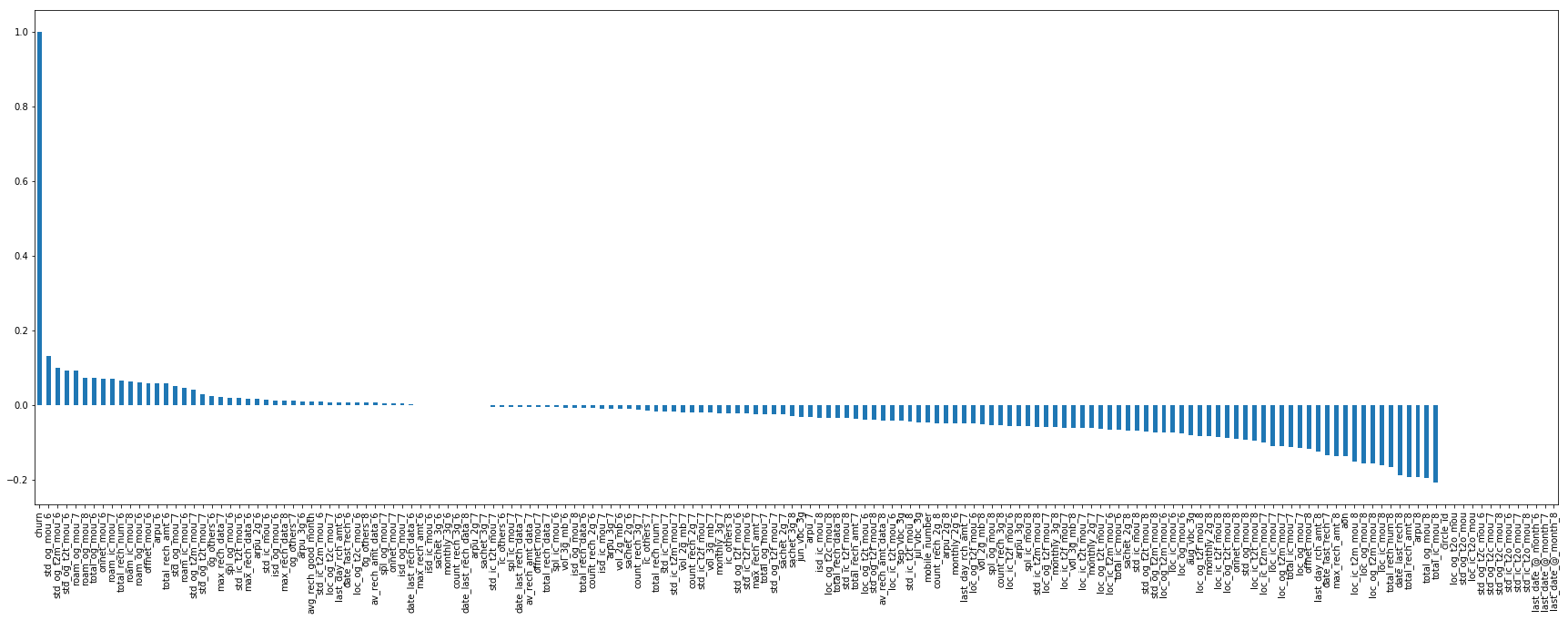In :
``````#plotting graph between Average revenue per user in july and Churn
ax = sns.distplot(df_high_end_cus['arpu_6'], hist=True, kde=False,
bins=int(180/5), color = 'darkblue',
hist_kws={'edgecolor':'black'},
kde_kws={'linewidth': 4})
ax.set_ylabel('Churn')
ax.set_xlabel('Average revenue per user in july')
ax.set_title('Relation between revenue and churn rate')``````
Out:
``Text(0.5, 1.0, 'Relation between revenue and churn rate')``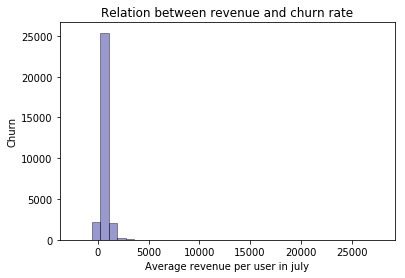In :
``````#plotting graph between Average revenue per user in August and Churn
ax = sns.distplot(df_high_end_cus['arpu_7'], hist=True, kde=False,
bins=int(180/5), color = 'darkblue',
hist_kws={'edgecolor':'black'},
kde_kws={'linewidth': 4})
ax.set_ylabel('Churn')
ax.set_xlabel('Average revenue per user in August')
ax.set_title('Relation between revenue and churn rate')``````
Out:
``Text(0.5, 1.0, 'Relation between revenue and churn rate')``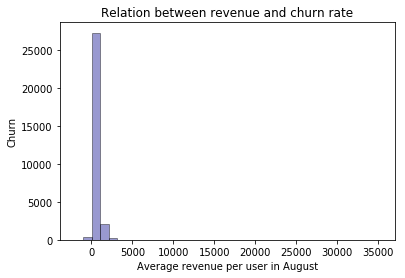In :
``````#dropping columns which are not helping in predicting churning
df_high_end_cus=df_high_end_cus.drop(columns=['mobile_number','circle_id'])``````

We can drop column `mobile_number`,`circle_id` as it does not help in predicting churning

In :
``````#correlation matrix
df_high_end_cus.corr()
``````
Out:

`We see above from the above heatmap that some of the correlation have Null Values(whitespaces) as these columns have same value and do not change, hence the standard deviation of these variables is 0 , which leads to Null values of correlation.`

##### Factor Analysis to check for multicollinearity
In :
``````#unsing FactorAnalysis from sklearn to estimate the maximum likelihood
from sklearn.decomposition import FactorAnalysis
FA = FactorAnalysis(n_components = 3).fit_transform(df_high_end_cus.values)``````
In :
``````#checking multicollinearity from the Factor Analysis Components using scatter plots
%matplotlib inline
import matplotlib.pyplot as plt
plt.figure(figsize=(12,8))
plt.title('Factor Analysis Components')
plt.scatter(FA[:,0], FA[:,1])
plt.scatter(FA[:,1], FA[:,2])
plt.scatter(FA[:,2], FA[:,0])``````
Out:
``<matplotlib.collections.PathCollection at 0x7fa11d293ed0>``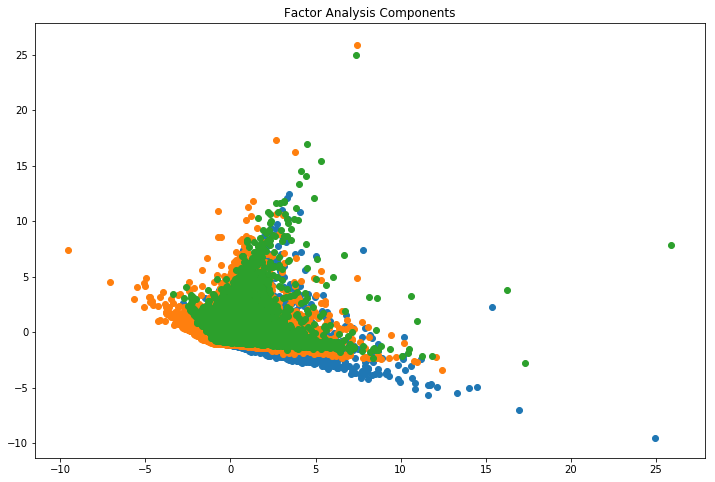We see that, the data is highly correlated and hence, we have to remove multicollinearity. Here, every group of factor is a set of highly correlated variables/columns

In :
``````#report = pp.ProfileReport(df_high_end_cus)
#report.to_file('output_report.html')``````

Dropping variables with `high multicollinearity` as per report from pandas-profile.

In :
``````df_high_end_cus = df_high_end_cus.drop(columns=['arpu_3g_6','arpu_3g_7','arpu_3g_8','isd_og_mou_7','isd_og_mou_8',
'sachet_2g_6','sachet_2g_7','sachet_2g_8','total_rech_amt_6',
'total_rech_amt_7','total_rech_amt_8'])``````

Dropping variables with `constant values` as per report from pandas-profile.

In :
``````df_high_end_cus = df_high_end_cus.drop(columns=['last_date_@_month_6','last_date_@_month_7','last_date_@_month_8',
'loc_ic_t2o_mou','loc_og_t2o_mou','std_ic_t2o_mou_6','std_ic_t2o_mou_7',
'std_ic_t2o_mou_8','std_og_t2c_mou_6','std_og_t2c_mou_7','std_og_t2c_mou_8',
'std_og_t2o_mou'])``````
In :
``df_high_end_cus.shape``
Out:
``(30011, 143)``

Now, identifying `skewed columns` and do the necessary transformations.

In :
``````sns.distplot(df_high_end_cus['avg_rech_good_month'])
plt.show()
``````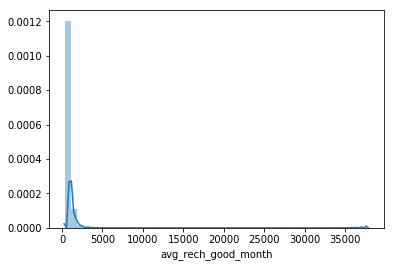In :
``#since, the column avg_rech_good_month is highly skewed, we can do log transformation.``
In :
``````df_high_end_cus['avg_rech_good_month_trnsfrm'] = np.log(df_high_end_cus['avg_rech_good_month'])
df_high_end_cus = df_high_end_cus.drop(columns=['avg_rech_good_month'])``````
In :
``````sns.distplot(df_high_end_cus['avg_rech_good_month_trnsfrm'])
plt.show()
``````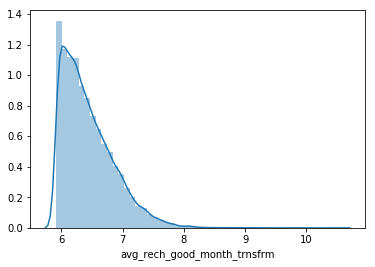In :
``````sns.distplot(df_high_end_cus['ic_others_6'])
plt.show()
``````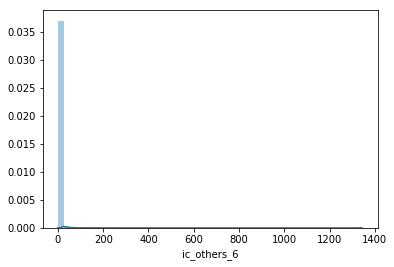In :
``````df_high_end_cus['ic_others_6_trnsfrm']=np.sqrt(df_high_end_cus['ic_others_6'])
df_high_end_cus['ic_others_8_trnsfrm']=np.sqrt(df_high_end_cus['ic_others_8'])
df_high_end_cus['isd_ic_mou_7_trnsfrm']=np.sqrt(df_high_end_cus['isd_ic_mou_7'])
df_high_end_cus['isd_ic_mou_6_trnsfrm']=np.sqrt(df_high_end_cus['isd_ic_mou_6'])
df_high_end_cus['loc_og_t2c_mou_7_trnsfrm']=np.sqrt(df_high_end_cus['loc_og_t2c_mou_7'])
df_high_end_cus['og_others_7_trnsfrm']=np.sqrt(df_high_end_cus['og_others_7'])
df_high_end_cus['og_others_8_trnsfrm']=np.sqrt(df_high_end_cus['og_others_8'])
df_high_end_cus['spl_ic_mou_6_trnsfrm']=np.sqrt(df_high_end_cus['spl_ic_mou_6'])
df_high_end_cus['spl_ic_mou_7_trnsfrm']=np.sqrt(df_high_end_cus['spl_ic_mou_7'])
df_high_end_cus['std_ic_t2f_mou_6_trnsfrm']=np.sqrt(df_high_end_cus['std_ic_t2f_mou_6'])
df_high_end_cus['std_ic_t2f_mou_7_trnsfrm']=np.sqrt(df_high_end_cus['std_ic_t2f_mou_7'])
df_high_end_cus['std_ic_t2f_mou_8_trnsfrm']=np.sqrt(df_high_end_cus['std_ic_t2f_mou_8'])
df_high_end_cus['std_ic_t2t_mou_6_trnsfrm']=np.sqrt(df_high_end_cus['std_ic_t2t_mou_6'])
df_high_end_cus['std_ic_t2t_mou_7_trnsfrm']=np.sqrt(df_high_end_cus['std_ic_t2t_mou_7'])
df_high_end_cus['std_ic_t2t_mou_8_trnsfrm']=np.sqrt(df_high_end_cus['std_ic_t2t_mou_8'])

cols_skewed_sqrt = ['ic_others_6','ic_others_8','isd_ic_mou_7','isd_ic_mou_6','loc_og_t2c_mou_7','og_others_7',
'og_others_8','spl_ic_mou_6','spl_ic_mou_7','std_ic_t2f_mou_6','std_ic_t2f_mou_7','std_ic_t2f_mou_8',
'std_ic_t2t_mou_6','std_ic_t2t_mou_7','std_ic_t2t_mou_8']

df_high_end_cus = df_high_end_cus.drop(columns=cols_skewed_sqrt,axis=1)``````
In :
``````#report_1 = pp.ProfileReport(df_high_end_cus)
#report_1.to_file('output_report_2.html')``````
In :
``````df_high_end_cus['ic_others_7_trnsfrm']=np.sqrt(df_high_end_cus['ic_others_7'])
df_high_end_cus['isd_og_mou_6_trnsfrm']=np.sqrt(df_high_end_cus['isd_og_mou_6'])

df_high_end_cus = df_high_end_cus.drop(columns=['ic_others_7','isd_og_mou_6'])

``````
In :
``FA_1 = FactorAnalysis(n_components = 3).fit_transform(df_high_end_cus.values)``
In :
``````#by plottig graph and visualizing the deduction of multicollinearity after dropping some columns
%matplotlib inline
import matplotlib.pyplot as plt
plt.figure(figsize=(12,8))
plt.title('Factor Analysis Components')
plt.scatter(FA_1[:,0],FA_1[:,1])
plt.scatter(FA_1[:,1], FA_1[:,2])
plt.scatter(FA_1[:,2],FA_1[:,0])
plt.show()

``````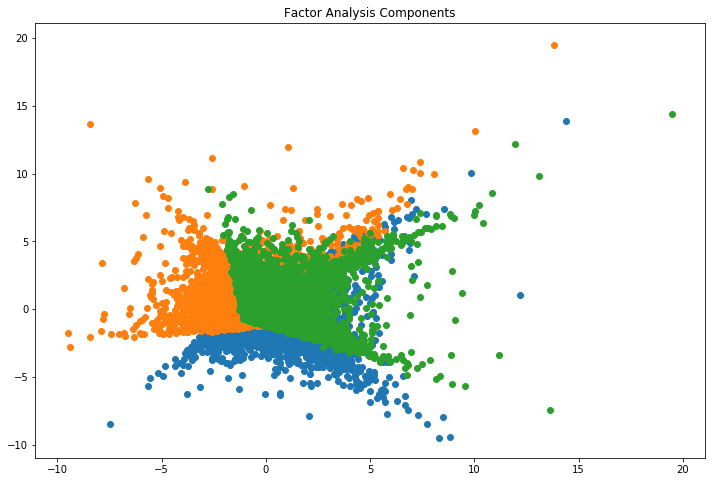In :
``````#report_2 = pp.ProfileReport(df_high_end_cus)
#report_2.to_file('output_report_3.html')``````

As we can see from the above factor plot, that the multicollinearity among the datasets have been reduced due to removal of the above mentioned columns as per pandas profiling.

In :
``````#Checking the correlation values in decending order
df_high_end_cus.corr()['churn'].sort_values(ascending = False)``````
Out:
``````churn                          1.000000
std_og_mou_6                   0.131846
std_og_t2m_mou_6               0.099027
std_og_t2t_mou_6               0.093168
roam_og_mou_7                  0.092717
roam_og_mou_8                  0.072746
total_og_mou_6                 0.072193
onnet_mou_6                    0.071193
roam_ic_mou_7                  0.069398
total_rech_num_6               0.064926
roam_ic_mou_8                  0.063417
roam_og_mou_6                  0.061005
offnet_mou_6                   0.058547
arpu_6                         0.058299
std_og_mou_7                   0.049341
roam_ic_mou_6                  0.045281
std_og_t2m_mou_7               0.039915
std_ic_t2t_mou_6_trnsfrm       0.032413
std_og_t2t_mou_7               0.029499
isd_og_mou_6_trnsfrm           0.027086
og_others_6                    0.023838
max_rech_data_7                0.021388
spl_og_mou_6                   0.019219
max_rech_data_6                0.016762
arpu_2g_6                      0.016439
std_ic_mou_6                   0.014432
max_rech_data_8                0.010959
std_ic_t2m_mou_6               0.009146
avg_rech_good_month_trnsfrm    0.007734
loc_og_t2c_mou_7_trnsfrm       0.007074
last_day_rch_amt_6             0.006301
date_last_rech_6               0.006051
loc_og_t2c_mou_6               0.005826
av_rech_amt_data_6             0.005406
spl_og_mou_7                   0.004479
onnet_mou_7                    0.004469
isd_ic_mou_6_trnsfrm           0.002928
og_others_7_trnsfrm            0.001932
date_last_rech_data_6          0.001892
max_rech_amt_6                 0.000408
sachet_3g_6                   -0.000659
monthly_3g_6                  -0.000746
count_rech_3g_6               -0.000943
date_last_rech_data_8         -0.001495
arpu_2g_7                     -0.001693
sachet_3g_7                   -0.001900
spl_ic_mou_7_trnsfrm          -0.004778
og_others_8_trnsfrm           -0.005165
date_last_rech_data_7         -0.005310
av_rech_amt_data_7            -0.005919
offnet_mou_7                  -0.005927
total_rech_data_7             -0.006471
std_ic_t2t_mou_7_trnsfrm      -0.006982
vol_3g_mb_6                   -0.007034
total_rech_data_6             -0.007746
count_rech_2g_6               -0.009005
vol_2g_mb_6                   -0.010459
ic_others_6_trnsfrm           -0.012010
count_rech_3g_7               -0.013208
spl_ic_mou_6_trnsfrm          -0.013247
total_rech_num_7              -0.017163
std_ic_mou_7                  -0.017219
std_ic_t2m_mou_7              -0.017647
isd_ic_mou_7_trnsfrm          -0.017897
vol_2g_mb_7                   -0.020292
count_rech_2g_7               -0.020302
vol_3g_mb_7                   -0.021298
monthly_3g_7                  -0.022068
std_og_t2f_mou_6              -0.023002
max_rech_amt_7                -0.025411
total_og_mou_7                -0.025698
std_og_t2f_mou_7              -0.025904
sachet_3g_8                   -0.030317
jun_vbc_3g                    -0.031576
arpu_7                        -0.032264
isd_ic_mou_8                  -0.033997
loc_og_t2c_mou_8              -0.035344
total_rech_data_8             -0.035385
ic_others_7_trnsfrm           -0.038166
loc_og_t2t_mou_6              -0.040706
std_og_t2f_mou_8              -0.040923
av_rech_amt_data_8            -0.041120
loc_ic_t2t_mou_6              -0.042433
sep_vbc_3g                    -0.043487
jul_vbc_3g                    -0.046368
count_rech_2g_8               -0.048568
arpu_2g_8                     -0.049299
monthly_2g_6                  -0.049323
last_day_rch_amt_7            -0.050486
loc_og_t2f_mou_6              -0.050514
vol_2g_mb_8                   -0.051534
std_ic_t2f_mou_6_trnsfrm      -0.051998
spl_og_mou_8                  -0.054581
count_rech_3g_8               -0.055715
loc_ic_t2f_mou_6              -0.056275
spl_ic_mou_8                  -0.057730
std_ic_t2f_mou_7_trnsfrm      -0.058405
std_ic_t2m_mou_8              -0.058697
loc_og_t2f_mou_7              -0.059601
monthly_3g_8                  -0.060327
loc_ic_t2f_mou_7              -0.060758
vol_3g_mb_8                   -0.061113
loc_ic_t2t_mou_7              -0.061258
monthly_2g_7                  -0.061820
loc_og_t2t_mou_7              -0.063534
loc_ic_t2m_mou_6              -0.065530
total_ic_mou_6                -0.065879
std_ic_mou_8                  -0.068913
std_og_t2t_mou_8              -0.072706
std_og_t2m_mou_8              -0.073009
ic_others_8_trnsfrm           -0.073157
loc_og_t2m_mou_6              -0.074936
loc_ic_mou_6                  -0.075223
loc_og_mou_6                  -0.076626
aug_vbc_3g                    -0.081413
loc_og_t2f_mou_8              -0.083690
monthly_2g_8                  -0.083851
loc_ic_t2f_mou_8              -0.085501
loc_og_t2t_mou_8              -0.089523
onnet_mou_8                   -0.090815
std_og_mou_8                  -0.092509
std_ic_t2t_mou_8_trnsfrm      -0.096043
loc_ic_t2t_mou_8              -0.097180
std_ic_t2f_mou_8_trnsfrm      -0.097314
loc_ic_t2m_mou_7              -0.101247
loc_ic_mou_7                  -0.110967
loc_og_t2m_mou_7              -0.111377
total_ic_mou_7                -0.113269
loc_og_mou_7                  -0.115236
offnet_mou_8                  -0.119262
last_day_rch_amt_8            -0.125655
date_last_rech_7              -0.134863
max_rech_amt_8                -0.136965
aon                           -0.138380
loc_ic_t2m_mou_8              -0.151994
loc_og_mou_8                  -0.156810
loc_og_t2m_mou_8              -0.157403
loc_ic_mou_8                  -0.163109
total_rech_num_8              -0.167651
date_last_rech_8              -0.190048
arpu_8                        -0.194774
total_og_mou_8                -0.195494
total_ic_mou_8                -0.207454
Name: churn, dtype: float64``````
In :
``````#using boxplot visualizing the outliers
sns.boxplot(df_high_end_cus['std_og_t2t_mou_6'])``````
Out:
``<matplotlib.axes._subplots.AxesSubplot at 0x7fa11cd5e250>``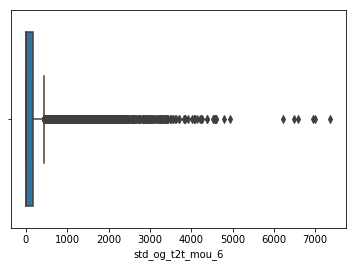In :
``df_high_end_cus.std_og_t2t_mou_6.quantile(0.99)``
Out:
``1885.1980000000017``
In :
``max(df_high_end_cus['std_og_t2t_mou_6'])``
Out:
``7366.58``

As we can see that for the column `std_og_t2t_mou_6`,there is a significant difference between the maximum value and the value of 99 th percentile, this shows there are clearly many outliers.

As a strategy to remove outliers, we retain values till 99th percentile of the top 10 correlated columns with column churn.

In :
``````churn_corr_cols = ['std_og_mou_6','std_og_t2m_mou_6','std_og_t2t_mou_6','roam_og_mou_7','roam_og_mou_8','total_og_mou_6',
'onnet_mou_6','roam_ic_mou_7','total_rech_num_6','roam_ic_mou_8']``````
In :
``````df_high_end_cus = df_high_end_cus[df_high_end_cus.std_og_mou_6 < df_high_end_cus.std_og_mou_6.quantile(.99)]
df_high_end_cus = df_high_end_cus[df_high_end_cus.std_og_t2m_mou_6 < df_high_end_cus.std_og_t2m_mou_6.quantile(.99)]
df_high_end_cus = df_high_end_cus[df_high_end_cus.std_og_t2t_mou_6 < df_high_end_cus.std_og_t2t_mou_6.quantile(.99)]
df_high_end_cus = df_high_end_cus[df_high_end_cus.roam_og_mou_7 < df_high_end_cus.roam_og_mou_7.quantile(.99)]
df_high_end_cus = df_high_end_cus[df_high_end_cus.roam_og_mou_8 < df_high_end_cus.roam_og_mou_8.quantile(.99)]
df_high_end_cus = df_high_end_cus[df_high_end_cus.total_og_mou_6 < df_high_end_cus.total_og_mou_6.quantile(.99)]
df_high_end_cus = df_high_end_cus[df_high_end_cus.onnet_mou_6 < df_high_end_cus.onnet_mou_6.quantile(.99)]
df_high_end_cus = df_high_end_cus[df_high_end_cus.roam_ic_mou_7 < df_high_end_cus.roam_ic_mou_7.quantile(.99)]
df_high_end_cus = df_high_end_cus[df_high_end_cus.total_rech_num_6 < df_high_end_cus.total_rech_num_6.quantile(.99)]
df_high_end_cus = df_high_end_cus[df_high_end_cus.roam_ic_mou_8 < df_high_end_cus.roam_ic_mou_8.quantile(.99)]

``````
In :
``````#after maximum possible outlier treatment
df_high_end_cus.shape
``````
Out:
``(27134, 143)``

#### Splitting into train - test data

In :
``````# split into X and y
X = df_high_end_cus.drop(columns=['churn'],axis=1)
y = df_high_end_cus['churn']``````
In :
``````# split into train and test with ratio of 80% and 20%
from sklearn.model_selection import train_test_split
X_train, X_test, y_train, y_test = train_test_split(X, y,
train_size=0.8,
test_size = 0.2, random_state=100)``````

#### Handling Class Imbalance

In :
``y_train.value_counts()``
Out:
``````0    19739
1     1968
Name: churn, dtype: int64``````
In :
``y_test.value_counts()``
Out:
``````0    4910
1     517
Name: churn, dtype: int64``````

As , we see there is a clear class imbalance of the customers who have churned and not churned. So, we apply methods like `SMOTE`(Synthetic Minority OverSampling Technique) to upsample the minority class of data points in `churn` column.

In :
``from imblearn.over_sampling import SMOTE``
In :
``````sm = SMOTE(random_state=27, ratio=1.0)
X_train, y_train = sm.fit_sample(X_train, y_train)
``````
In :
``np.bincount(y_train) #19739 rows of each class for the column churn``
Out:
``array([19739, 19739])``
In :
``````# Converting n-arrays to dataframe
X_train_df = pd.DataFrame(X_train)
y_train_df = pd.DataFrame(y_train)
``````
In :
``X_train_df.columns = X.columns``

#### Feature Scaling

In :
``from sklearn.preprocessing import StandardScaler``
In :
``````scaler = StandardScaler()

X_train_df_scaled = scaler.fit_transform(X_train_df)
``````

### Model Building and Evaluation

Ridge and Lasso Regression Let's now try predicting churn customers with ridge and lasso regression.

### Ridge Regression

In :
``````# list of alphas to tune
params = {'alpha': [0.0001, 0.001, 0.01, 0.05, 0.1,
0.2, 0.3, 0.4, 0.5, 0.6, 0.7, 0.8, 0.9, 1.0, 2.0, 3.0,
4.0, 5.0, 6.0, 7.0, 8.0, 9.0, 10.0, 20, 50, 100, 500, 1000 ]}

# Applying Ridge
ridge = Ridge()

# cross validation
folds = 5
model_cv = GridSearchCV(estimator = ridge,
param_grid = params,
scoring= 'neg_mean_absolute_error',
cv = folds,
return_train_score=True,
verbose = 1)
model_cv.fit(X_train_df_scaled, y_train_df)
``````
```Fitting 5 folds for each of 28 candidates, totalling 140 fits ```
```[Parallel(n_jobs=1)]: Using backend SequentialBackend with 1 concurrent workers. [Parallel(n_jobs=1)]: Done 140 out of 140 | elapsed: 12.9s finished ```
Out:
``````GridSearchCV(cv=5, error_score='raise-deprecating',
estimator=Ridge(alpha=1.0, copy_X=True, fit_intercept=True,
max_iter=None, normalize=False, random_state=None,
solver='auto', tol=0.001),
iid='warn', n_jobs=None,
param_grid={'alpha': [0.0001, 0.001, 0.01, 0.05, 0.1, 0.2, 0.3,
0.4, 0.5, 0.6, 0.7, 0.8, 0.9, 1.0, 2.0, 3.0,
4.0, 5.0, 6.0, 7.0, 8.0, 9.0, 10.0, 20, 50,
100, 500, 1000]},
pre_dispatch='2*n_jobs', refit=True, return_train_score=True,
scoring='neg_mean_absolute_error', verbose=1)``````
In :
``````cv_results = pd.DataFrame(model_cv.cv_results_)
cv_results = cv_results[cv_results['param_alpha']<=200]
cv_results.head()
``````
Out:
In :
``````# plotting mean test and train scoes with alpha
cv_results['param_alpha'] = cv_results['param_alpha'].astype('int32')

# plotting
plt.plot(cv_results['param_alpha'], cv_results['mean_train_score'])
plt.plot(cv_results['param_alpha'], cv_results['mean_test_score'])
plt.xlabel('alpha')
plt.ylabel('Negative Mean Absolute Error')
plt.title("Negative Mean Absolute Error and alpha")
plt.legend(['train score', 'test score'], loc='upper left')
plt.show()
``````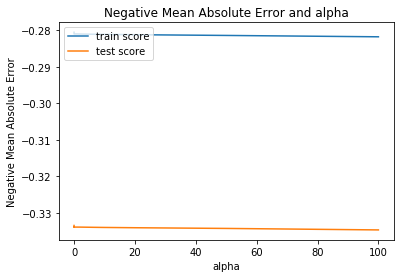In :
``````alpha = 15
ridge = Ridge(alpha=alpha)

ridge.fit(X_train, y_train)
ridge.coef_
``````
Out:
``````array([ 3.33557838e-05,  6.99107024e-05, -2.74112464e-05, -4.69233322e-05,
-2.70647164e-04,  1.11016372e-03,  8.81463225e-05,  8.51246713e-05,
5.72169364e-04, -6.03432246e-05,  3.39585575e-04,  7.17411351e-04,
7.94907451e-05,  1.96781340e-04,  5.10217696e-04,  5.37262773e-02,
5.61354468e-02,  6.46483949e-02,  5.36048108e-02,  5.57673459e-02,
6.52130118e-02,  5.35700597e-02,  5.56777588e-02,  6.49154341e-02,
-1.01251135e-03, -4.18832794e-06, -5.29493666e-02, -5.56037003e-02,
-6.56510960e-02, -3.73706126e-02, -4.61414201e-02, -9.40563614e-02,
-3.75697987e-02, -4.64150333e-02, -9.35520597e-02, -3.71726651e-02,
-4.72984839e-02, -9.38964488e-02,  3.82022585e-02,  4.66900023e-02,
9.31680487e-02,  1.08691964e-03,  3.05819335e-04, -4.89244687e-04,
-9.63052546e-04, -6.91880316e-04, -2.42218816e-04, -3.12840619e-04,
4.52916823e-02,  8.38720584e-02,  9.43816815e-02,  4.52305948e-02,
8.39614900e-02,  9.43082800e-02,  4.51242156e-02,  8.39545944e-02,
9.43958243e-02, -4.51798611e-02, -8.38325530e-02, -9.52499473e-02,
1.62102950e-04,  2.39288431e-06, -6.44145124e-04, -9.66700616e-05,
-8.53345486e-05, -8.54989355e-05, -5.30885415e-05,  3.43607501e-05,
5.90231257e-04, -1.91500478e-01, -7.92869488e-04,  2.01061352e-03,
5.14819917e-03, -9.87424630e-03,  2.19103830e-05,  1.93633287e-05,
6.10984278e-05,  6.07772347e-05, -3.03909391e-05, -5.41330740e-04,
3.31001044e-03,  8.97130176e-03,  1.35735657e-01,  3.78181416e-06,
3.37212319e-04, -6.67238323e-04,  2.09548113e-05, -1.04714642e-02,
-1.34843113e-01,  3.28905564e-03,  7.79189242e-03, -1.27317320e-01,
-4.61116348e-05, -1.26881628e-04,  6.51182268e-05,  1.57715578e-05,
5.81945540e-05, -3.79725587e-05, -5.89433676e-06,  2.56943595e-05,
-2.13470023e-06, -6.37788489e-06, -6.57527087e-05,  5.35091788e-04,
-2.40085739e-02, -1.50330256e-02, -8.59655238e-03,  5.54380048e-03,
1.74598671e-02, -9.89796282e-02, -2.25474485e-03, -9.66797469e-03,
-2.83376920e-02, -6.33096436e-05, -5.63542647e-05,  3.84441427e-06,
6.14315310e-06, -3.74902909e-04, -1.54191461e-03,  1.99958363e-03,
-1.64346525e-03, -2.25151189e-04, -1.72247717e-03, -1.50039593e-02,
-5.35952605e-02, -4.59318666e-03, -1.50141651e-02,  4.87805960e-03,
6.79322982e-04,  5.58799437e-03,  8.53584851e-03, -1.45473867e-02,
-7.43729578e-03, -3.57948894e-02,  2.89562258e-03,  6.76398574e-03,
-2.60608273e-02,  6.59043173e-03,  1.07475303e-02, -2.87028112e-02,
3.77968345e-03,  1.39464959e-02])``````
In :
``#storing the assigned coefficients from ridge regression in an array``
In :
``coef_ridge_array = ridge.coef_``
In :
``df_ridge_feature_select = pd.DataFrame(coef_ridge_array,X_train_df.columns)``
In :
``df_ridge_feature_select.columns=['coefficient']``
In :
``top5features_ridge = sorted(coef_ridge_array,reverse = True)[:5]``
In :
``df_ridge_feature_select.loc[df_ridge_feature_select['coefficient'].isin(top5features)]``
Out:
In :
``y_train_pred = ridge.predict(X_train_df).reshape(-1)``
In :
``y_train_pred``
Out:
``````array([ 0.24752928, -0.19069981,  0.32454788, ...,  0.93995485,
0.52469585,  0.70365213])``````
In :
``y_train_pred_final = pd.DataFrame({'Churn':y_train, 'Churn_Prob':y_train_pred})``
In :
``y_train_pred_final['predicted'] = y_train_pred_final.Churn_Prob.map(lambda x: 1 if x > 0.5 else 0)``
In :
``y_train_pred_final.head()``
Out:
In :
``````# Let's check the overall accuracy.
from sklearn import metrics
print(metrics.accuracy_score(y_train_pred_final.Churn, y_train_pred_final.predicted))
``````
```0.8568316530725973 ```

#### ROC Curve

In :
``````def draw_roc( actual, probs ):
fpr, tpr, thresholds = metrics.roc_curve( actual, probs,
drop_intermediate = False )
auc_score = metrics.roc_auc_score( actual, probs )
plt.figure(figsize=(5, 5))
plt.plot( fpr, tpr, label='ROC curve (area = %0.2f)' % auc_score )
plt.plot([0, 1], [0, 1], 'k--')
plt.xlim([0.0, 1.0])
plt.ylim([0.0, 1.05])
plt.xlabel('False Positive Rate or [1 - True Negative Rate]')
plt.ylabel('True Positive Rate')
plt.title('Receiver operating characteristic example')
plt.legend(loc="lower right")
plt.show()

return None``````
In :
``fpr, tpr, thresholds = metrics.roc_curve( y_train_pred_final.Churn, y_train_pred_final.Churn_Prob, drop_intermediate = False )``
In :
``draw_roc(y_train_pred_final.Churn, y_train_pred_final.Churn_Prob)``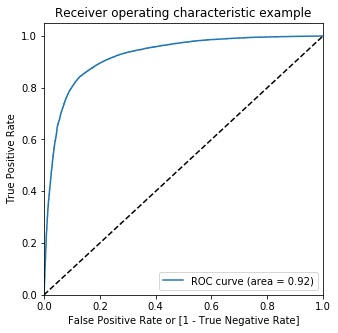#### Finding Optimal cut-off point

In :
``````numbers = [float(x)/10 for x in range(10)]
for i in numbers:
y_train_pred_final[i]= y_train_pred_final.Churn_Prob.map(lambda x: 1 if x > i else 0)
y_train_pred_final.head()
``````
Out:
In :
``````# Now let's calculate accuracy sensitivity and specificity for various probability cutoffs.
cutoff_df = pd.DataFrame( columns = ['prob','accuracy','sensi','speci'])
from sklearn.metrics import confusion_matrix

# TP = confusion[1,1] # true positive
# TN = confusion[0,0] # true negatives
# FP = confusion[0,1] # false positives
# FN = confusion[1,0] # false negatives

num = [0.0,0.1,0.2,0.3,0.4,0.5,0.6,0.7,0.8,0.9]
for i in num:
cm1 = metrics.confusion_matrix(y_train_pred_final.Churn, y_train_pred_final[i] )
total1=sum(sum(cm1))
accuracy = (cm1[0,0]+cm1[1,1])/total1

speci = cm1[0,0]/(cm1[0,0]+cm1[0,1])
sensi = cm1[1,1]/(cm1[1,0]+cm1[1,1])
cutoff_df.loc[i] =[ i ,accuracy,sensi,speci]
print(cutoff_df)
``````
``` prob accuracy sensi speci 0.0 0.0 0.568798 0.997518 0.140078 0.1 0.1 0.621080 0.994731 0.247429 0.2 0.2 0.694539 0.985967 0.403111 0.3 0.3 0.773950 0.961042 0.586859 0.4 0.4 0.836390 0.917017 0.755763 0.5 0.5 0.856832 0.843964 0.869700 0.6 0.6 0.832058 0.734992 0.929125 0.7 0.7 0.776559 0.592381 0.960738 0.8 0.8 0.702847 0.427732 0.977962 0.9 0.9 0.638102 0.287705 0.988500 ```
In :
``````# Let's plot accuracy sensitivity and specificity for various probabilities.
cutoff_df.plot.line(x='prob', y=['accuracy','sensi','speci'])
plt.show()
``````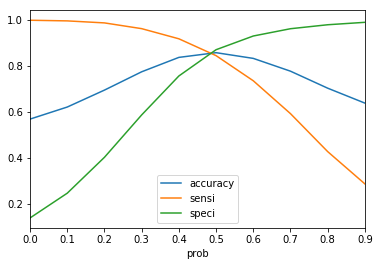Thus , from the cutoff it seems that `0.5` is a good cutoff point for the ridge regularisation.
Accuracy obtained from the lasso regularisation --> `85.1%`
Also , we see that the sensitivity/recall is around `84.3 %` when cut off probability is around 0.5

### Lasso Regression

In :
``````# Applying Lasso
lasso = Lasso()

# cross validation
model_cv = GridSearchCV(estimator = lasso,
param_grid = params,
scoring= 'neg_mean_absolute_error',
cv = folds,
return_train_score=True,
verbose = 1)

model_cv.fit(X_train_df_scaled, y_train_df)
``````
```Fitting 5 folds for each of 28 candidates, totalling 140 fits ```
```[Parallel(n_jobs=1)]: Using backend SequentialBackend with 1 concurrent workers. [Parallel(n_jobs=1)]: Done 140 out of 140 | elapsed: 1.2min finished ```
Out:
``````GridSearchCV(cv=5, error_score='raise-deprecating',
estimator=Lasso(alpha=1.0, copy_X=True, fit_intercept=True,
max_iter=1000, normalize=False, positive=False,
precompute=False, random_state=None,
selection='cyclic', tol=0.0001, warm_start=False),
iid='warn', n_jobs=None,
param_grid={'alpha': [0.0001, 0.001, 0.01, 0.05, 0.1, 0.2, 0.3,
0.4, 0.5, 0.6, 0.7, 0.8, 0.9, 1.0, 2.0, 3.0,
4.0, 5.0, 6.0, 7.0, 8.0, 9.0, 10.0, 20, 50,
100, 500, 1000]},
pre_dispatch='2*n_jobs', refit=True, return_train_score=True,
scoring='neg_mean_absolute_error', verbose=1)``````
In :
``````cv_results = pd.DataFrame(model_cv.cv_results_)
cv_results.head()
``````
Out:
In :
``````# plotting mean test and train scoes with alpha
cv_results['param_alpha'] = cv_results['param_alpha'].astype('float32')

# plotting
plt.plot(cv_results['param_alpha'], cv_results['mean_train_score'])
plt.plot(cv_results['param_alpha'], cv_results['mean_test_score'])
plt.xlabel('alpha')
plt.ylabel('Negative Mean Absolute Error')

plt.title("Negative Mean Absolute Error and alpha")
plt.legend(['train score', 'test score'], loc='upper left')
plt.show()
``````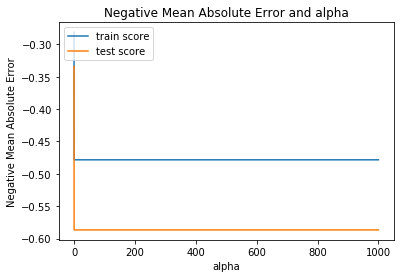In :
``````alpha =100

lasso = Lasso(alpha=alpha)

lasso.fit(X_train, y_train)
``````
Out:
``````Lasso(alpha=100, copy_X=True, fit_intercept=True, max_iter=1000,
normalize=False, positive=False, precompute=False, random_state=None,
selection='cyclic', tol=0.0001, warm_start=False)``````
In :
``lasso.coef_``
Out:
``````array([ 0.00000000e+00, -0.00000000e+00, -0.00000000e+00,  0.00000000e+00,
-0.00000000e+00, -0.00000000e+00,  0.00000000e+00, -0.00000000e+00,
-0.00000000e+00, -0.00000000e+00,  0.00000000e+00,  0.00000000e+00,
0.00000000e+00,  0.00000000e+00,  0.00000000e+00, -0.00000000e+00,
-0.00000000e+00, -0.00000000e+00, -0.00000000e+00, -0.00000000e+00,
-0.00000000e+00, -0.00000000e+00, -0.00000000e+00, -0.00000000e+00,
0.00000000e+00, -0.00000000e+00, -0.00000000e+00, -0.00000000e+00,
-0.00000000e+00,  0.00000000e+00,  0.00000000e+00, -0.00000000e+00,
0.00000000e+00,  0.00000000e+00, -0.00000000e+00, -0.00000000e+00,
-0.00000000e+00, -0.00000000e+00,  0.00000000e+00,  0.00000000e+00,
-0.00000000e+00,  0.00000000e+00, -0.00000000e+00, -0.00000000e+00,
0.00000000e+00,  0.00000000e+00, -0.00000000e+00, -6.90712746e-06,
-0.00000000e+00, -0.00000000e+00, -0.00000000e+00, -0.00000000e+00,
-0.00000000e+00, -0.00000000e+00, -0.00000000e+00, -0.00000000e+00,
-0.00000000e+00, -0.00000000e+00, -0.00000000e+00, -0.00000000e+00,
-0.00000000e+00, -0.00000000e+00, -0.00000000e+00, -0.00000000e+00,
-0.00000000e+00, -0.00000000e+00, -0.00000000e+00, -0.00000000e+00,
-0.00000000e+00, -0.00000000e+00, -0.00000000e+00,  0.00000000e+00,
-0.00000000e+00, -0.00000000e+00, -0.00000000e+00, -0.00000000e+00,
-0.00000000e+00,  0.00000000e+00, -0.00000000e+00, -0.00000000e+00,
-0.00000000e+00, -0.00000000e+00, -0.00000000e+00,  0.00000000e+00,
0.00000000e+00,  0.00000000e+00, -0.00000000e+00, -0.00000000e+00,
-0.00000000e+00,  0.00000000e+00, -0.00000000e+00, -0.00000000e+00,
0.00000000e+00, -0.00000000e+00, -0.00000000e+00, -0.00000000e+00,
-0.00000000e+00, -0.00000000e+00, -0.00000000e+00, -0.00000000e+00,
-0.00000000e+00,  0.00000000e+00,  0.00000000e+00, -0.00000000e+00,
-0.00000000e+00, -0.00000000e+00, -0.00000000e+00,  0.00000000e+00,
-0.00000000e+00, -0.00000000e+00,  0.00000000e+00, -0.00000000e+00,
-0.00000000e+00, -2.81589424e-05, -0.00000000e+00, -0.00000000e+00,
-0.00000000e+00, -0.00000000e+00,  0.00000000e+00,  0.00000000e+00,
0.00000000e+00,  0.00000000e+00, -0.00000000e+00, -0.00000000e+00,
-0.00000000e+00, -0.00000000e+00, -0.00000000e+00, -0.00000000e+00,
0.00000000e+00, -0.00000000e+00,  0.00000000e+00, -0.00000000e+00,
-0.00000000e+00, -0.00000000e+00, -0.00000000e+00, -0.00000000e+00,
-0.00000000e+00,  0.00000000e+00, -0.00000000e+00, -0.00000000e+00,
-0.00000000e+00,  0.00000000e+00])``````
In :
``coef_lasso_array = lasso.coef_``
In :
``df_lasso_feature_select = pd.DataFrame(coef_lasso_array,X_train_df.columns)``
In :
``df_lasso_feature_select.columns=['coefficient']``
In :
``top5features_lasso = sorted(coef_lasso_array,reverse = True)[:5]``
In :
``df_lasso_feature_select.loc[df_lasso_feature_select['coefficient'].isin(top5features_lasso)]``
Out:

As we see above that most of the features have been nullified by lasso regularisation, so it is better to use ridge regression for feature selection.

### Logistic Regression

Model with simple `logistic regression` using `SAGA (Stochastic Average Gradient descent solver)` that includes both L1 and L2 regularisation.

In :
``smote = LogisticRegression(solver='saga').fit(X_train_df_scaled, y_train_df)``
In :
``X_test = scaler.transform(X_test)``
In :
``````smote_pred = smote.predict(X_test)

``````
In :
``````print(accuracy_score(y_test, smote_pred))
#0.84

# f1 score
print(f1_score(y_test, smote_pred))
#0.51

print(recall_score(y_test, smote_pred))
#0.83

print(precision_score(y_test, smote_pred))
#0.37``````
```0.8494564215957251 0.5145573380867499 0.8375241779497099 0.3713550600343053 ```

#### Logistic Regression with PCA

In :
``````from sklearn.decomposition import PCA
from sklearn.metrics import mean_squared_error, r2_score
from sklearn.metrics import explained_variance_score``````
In :
``pca = PCA(n_components=40,random_state=100,svd_solver='randomized')``
In :
``````Xtrain_reduced = pca.fit_transform(X_train_df_scaled)
Xtest_reduced = pca.transform(X_test)

regrpca = LogisticRegression()

# Train the model using the principal components of the transformed training sets
regrpca.fit(Xtrain_reduced, y_train_df)
# Make predictions using the principal components of the transformed testing set
y_pred = regrpca.predict(Xtest_reduced)
print("Mean squared error: %.2f" % mean_squared_error(y_test, y_pred))
# Explained variance score: 1 is perfect prediction
print('R2 score: %.2f' % r2_score(y_test, y_pred))

``````
```Mean squared error: 0.19 R2 score: -1.15 ```
In :
``sum(pca.explained_variance_ratio_)``
Out:
``0.7986403605500407``
In :
``````#plotting a scree plot
fig = plt.figure(figsize = (12,8))
plt.plot(np.cumsum(pca.explained_variance_ratio_))
plt.xlabel('number of components')
plt.ylabel('cumulative explained variance')
plt.show()
``````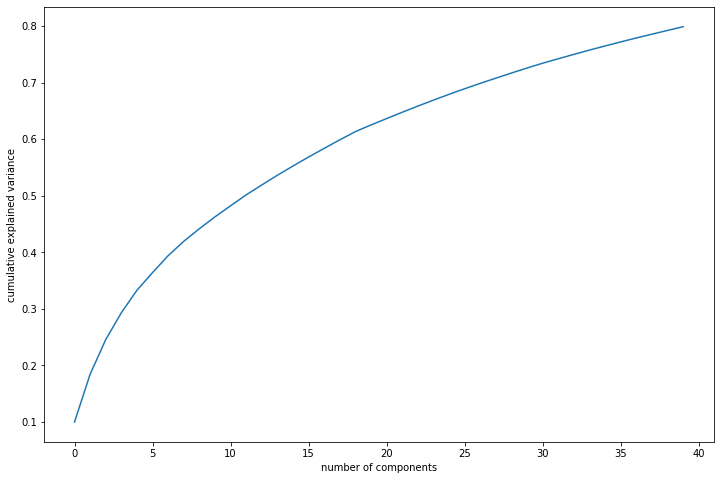In :
``````print(accuracy_score(y_test, y_pred))
#0.76

# f1 score
print(f1_score(y_test, y_pred))
#0.393

print(recall_score(y_test, y_pred))
#0.814

print(precision_score(y_test, y_pred))
#0.259``````
```0.8148148148148148 0.45646295294753925 0.816247582205029 0.31681681681681684 ```

We see that the accuracy scores, precision,recall scores are pretty low for logistic regression model with PCA components

### Random Forest Classifier with PCA Components

Let's start with the default hyperparameters

In :
``````# Importing random forest classifier from sklearn library
from sklearn.ensemble import RandomForestClassifier

# Running the random forest with default parameters.
rfc = RandomForestClassifier()
``````
In :
``````# fit
rfc.fit(Xtrain_reduced,y_train)
``````
Out:
``````RandomForestClassifier(bootstrap=True, class_weight=None, criterion='gini',
max_depth=None, max_features='auto', max_leaf_nodes=None,
min_impurity_decrease=0.0, min_impurity_split=None,
min_samples_leaf=1, min_samples_split=2,
min_weight_fraction_leaf=0.0, n_estimators=10,
n_jobs=None, oob_score=False, random_state=None,
verbose=0, warm_start=False)``````
In :
``````# Making predictions
predictions = rfc.predict(Xtest_reduced)
``````
In :
``````# Importing classification report and confusion matrix from sklearn metrics
from sklearn.metrics import classification_report,confusion_matrix, accuracy_score``````
In :
``````# Let's check the report of our default model
print(classification_report(y_test,predictions))
``````
``` precision recall f1-score support 0 0.95 0.93 0.94 4910 1 0.45 0.51 0.48 517 accuracy 0.89 5427 macro avg 0.70 0.72 0.71 5427 weighted avg 0.90 0.89 0.90 5427 ```
In :
``````# Printing confusion matrix
print(confusion_matrix(y_test,predictions))
``````
```[[4587 323] [ 253 264]] ```
In :
``print(accuracy_score(y_test,predictions))``
```0.8938640132669984 ```

We see that we get a accuracy of `88.8%` , average precision of `69 %` , recall of `73 %` if we use random forest model with PCA Components.

##### Tuning Hyperparameters

Trying to find the optimum values for max_depth and understand how the value of max_depth impacts the overall accuracy of the ensemble.

###### Tuning max_Depth
In :
``````# GridSearchCV to find optimal n_estimators
from sklearn.model_selection import KFold
from sklearn.model_selection import GridSearchCV

# specify number of folds for k-fold CV
n_folds = 5

# parameters to build the model on
parameters = {'max_depth': range(2, 20, 5)}

# instantiate the model
rf = RandomForestClassifier()

# fit tree on training data
rf = GridSearchCV(rf, parameters,
cv=n_folds,
scoring="accuracy",return_train_score=True)
rf.fit(Xtrain_reduced, y_train)
``````
Out:
``````GridSearchCV(cv=5, error_score='raise-deprecating',
estimator=RandomForestClassifier(bootstrap=True, class_weight=None,
criterion='gini', max_depth=None,
max_features='auto',
max_leaf_nodes=None,
min_impurity_decrease=0.0,
min_impurity_split=None,
min_samples_leaf=1,
min_samples_split=2,
min_weight_fraction_leaf=0.0,
n_estimators='warn', n_jobs=None,
oob_score=False,
random_state=None, verbose=0,
warm_start=False),
iid='warn', n_jobs=None, param_grid={'max_depth': range(2, 20, 5)},
pre_dispatch='2*n_jobs', refit=True, return_train_score=True,
scoring='accuracy', verbose=0)``````
In :
``````# scores of GridSearch CV
scores = rf.cv_results_
pd.DataFrame(scores).head()
``````
Out:
In :
``````# plotting accuracies with max_depth
plt.figure()
plt.plot(scores["param_max_depth"],
scores["mean_train_score"],
label="training accuracy")
plt.plot(scores["param_max_depth"],
scores["mean_test_score"],
label="test accuracy")
plt.xlabel("max_depth")
plt.ylabel("Accuracy")
plt.legend()
plt.show()

``````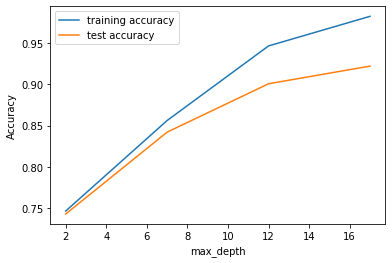Thus , we see that the above a certain point the depth if increased the training accuracy and test accuracy seems to grow at a steady rate. You can see that as we increase the value of max_depth, both train and test scores increase till a point, but after that test score starts to decrease. The ensemble tries to overfit as we increase the max_depth.

Thus, controlling the depth of the constituent trees will help reduce overfitting in the forest.

###### Tuning n_estimators

Finding the optimum values for n_estimators and understand how the value of n_estimators impacts the overall accuracy.We will try to provide appropriately low value of max_depth, so that the trees do not overfit.

In :
``````# GridSearchCV to find optimal n_estimators
from sklearn.model_selection import KFold
from sklearn.model_selection import GridSearchCV

# specify number of folds for k-fold CV
n_folds = 5

# parameters to build the model on
parameters = {'n_estimators': range(20, 100, 10)}

# instantiate the model (note we are specifying a max_depth)
rf = RandomForestClassifier(max_depth=6)

# fit tree on training data
rf = GridSearchCV(rf, parameters,
cv=n_folds,
scoring="accuracy",return_train_score=True)
rf.fit(Xtrain_reduced, y_train)
``````
Out:
``````GridSearchCV(cv=5, error_score='raise-deprecating',
estimator=RandomForestClassifier(bootstrap=True, class_weight=None,
criterion='gini', max_depth=6,
max_features='auto',
max_leaf_nodes=None,
min_impurity_decrease=0.0,
min_impurity_split=None,
min_samples_leaf=1,
min_samples_split=2,
min_weight_fraction_leaf=0.0,
n_estimators='warn', n_jobs=None,
oob_score=False,
random_state=None, verbose=0,
warm_start=False),
iid='warn', n_jobs=None,
param_grid={'n_estimators': range(20, 100, 10)},
pre_dispatch='2*n_jobs', refit=True, return_train_score=True,
scoring='accuracy', verbose=0)``````
In :
``````# scores of GridSearch CV
scores = rf.cv_results_
pd.DataFrame(scores).head()
``````
Out:
In :
``````# plotting accuracies with n_estimators
plt.figure()
plt.plot(scores["param_n_estimators"],
scores["mean_train_score"],
label="training accuracy")
plt.plot(scores["param_n_estimators"],
scores["mean_test_score"],
label="test accuracy")
plt.xlabel("n_estimators")
plt.ylabel("Accuracy")
plt.legend()
plt.show()

``````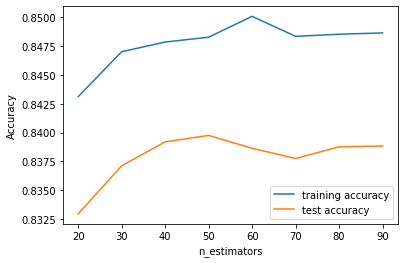We see that optimal value of n_estimators would be iin range of 60 to 90.

###### Tuning max_features

Model performance varies with max_features, which is the maximum numbre of features considered for splitting at a node.

In :
``````# GridSearchCV to find optimal max_features
from sklearn.model_selection import KFold
from sklearn.model_selection import GridSearchCV

# specify number of folds for k-fold CV
n_folds = 5

# parameters to build the model on
parameters = {'max_features': [4, 8, 14, 20, 24]}

# instantiate the model
rf = RandomForestClassifier(max_depth=6)

# fit tree on training data
rf = GridSearchCV(rf, parameters,
cv=n_folds,
scoring="accuracy",return_train_score=True)
rf.fit(Xtrain_reduced, y_train)
``````
Out:
``````GridSearchCV(cv=5, error_score='raise-deprecating',
estimator=RandomForestClassifier(bootstrap=True, class_weight=None,
criterion='gini', max_depth=6,
max_features='auto',
max_leaf_nodes=None,
min_impurity_decrease=0.0,
min_impurity_split=None,
min_samples_leaf=1,
min_samples_split=2,
min_weight_fraction_leaf=0.0,
n_estimators='warn', n_jobs=None,
oob_score=False,
random_state=None, verbose=0,
warm_start=False),
iid='warn', n_jobs=None,
param_grid={'max_features': [4, 8, 14, 20, 24]},
pre_dispatch='2*n_jobs', refit=True, return_train_score=True,
scoring='accuracy', verbose=0)``````
In :
``````# scores of GridSearch CV
scores = rf.cv_results_
pd.DataFrame(scores).head()
``````
Out:
In :
``````# plotting accuracies with max_features
plt.figure()
plt.plot(scores["param_max_features"],
scores["mean_train_score"],
label="training accuracy")
plt.plot(scores["param_max_features"],
scores["mean_test_score"],
label="test accuracy")
plt.xlabel("max_features")
plt.ylabel("Accuracy")
plt.legend()
plt.show()

``````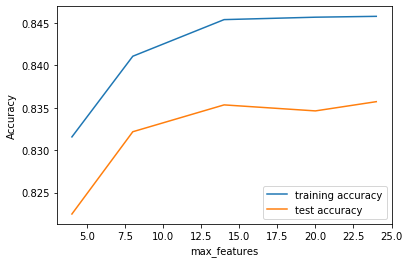We see that , the number of maximum features that should be present is between 15 to 20. Apparently, the training and test scores both seem to increase as we increase max_features, and the model doesn't seem to overfit more with increasing max_features. Think about why that might be the case.

#### Tuning min_samples_split

The hyperparameter min_samples_leaf is the minimum number of samples required to be at a leaf node:

If int, then consider min_samples_leaf as the minimum number. If float, then min_samples_leaf is a percentage and ceil(min_samples_leaf * n_samples) are the minimum number of samples for each node.

Let's now look at the performance of the ensemble as we vary min_samples_split.

In :
``````# GridSearchCV to find optimal min_samples_split
from sklearn.model_selection import KFold
from sklearn.model_selection import GridSearchCV

# specify number of folds for k-fold CV
n_folds = 5

# parameters to build the model on
parameters = {'min_samples_split': range(10, 200, 50)}

# instantiate the model
rf = RandomForestClassifier()

# fit tree on training data
rf = GridSearchCV(rf, parameters,
cv=n_folds,
scoring="accuracy",return_train_score=True)
rf.fit(Xtrain_reduced, y_train)
``````
Out:
``````GridSearchCV(cv=5, error_score='raise-deprecating',
estimator=RandomForestClassifier(bootstrap=True, class_weight=None,
criterion='gini', max_depth=None,
max_features='auto',
max_leaf_nodes=None,
min_impurity_decrease=0.0,
min_impurity_split=None,
min_samples_leaf=1,
min_samples_split=2,
min_weight_fraction_leaf=0.0,
n_estimators='warn', n_jobs=None,
oob_score=False,
random_state=None, verbose=0,
warm_start=False),
iid='warn', n_jobs=None,
param_grid={'min_samples_split': range(10, 200, 50)},
pre_dispatch='2*n_jobs', refit=True, return_train_score=True,
scoring='accuracy', verbose=0)``````
In :
``````# scores of GridSearch CV
scores = rf.cv_results_
pd.DataFrame(scores).head()
``````
Out:
In :
``````# plotting accuracies with min_samples_split
plt.figure()
plt.plot(scores["param_min_samples_split"],
scores["mean_train_score"],
label="training accuracy")
plt.plot(scores["param_min_samples_split"],
scores["mean_test_score"],
label="test accuracy")
plt.xlabel("min_samples_split")
plt.ylabel("Accuracy")
plt.legend()
plt.show()
``````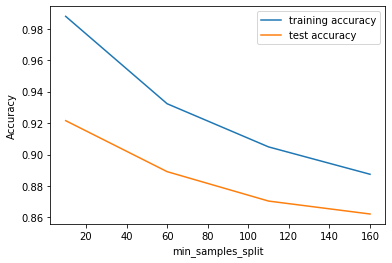We see that the accuracy goes down as the number of samples per split increases and the model starts of overfit as we decrease the value of min_samples_leaf.

##### Tuning min_samples_leaf

Let's now look at the performance of the ensemble as we vary min_samples_split.

In :
``````# GridSearchCV to find optimal min_samples_split
from sklearn.model_selection import KFold
from sklearn.model_selection import GridSearchCV

# specify number of folds for k-fold CV
n_folds = 5

# parameters to build the model on
parameters = {'min_samples_leaf': range(200, 500, 50)}

# instantiate the model
rf = RandomForestClassifier()

# fit tree on training data
rf = GridSearchCV(rf, parameters,
cv=n_folds,
scoring="accuracy",return_train_score=True)
rf.fit(Xtrain_reduced, y_train)
``````
Out:
``````GridSearchCV(cv=5, error_score='raise-deprecating',
estimator=RandomForestClassifier(bootstrap=True, class_weight=None,
criterion='gini', max_depth=None,
max_features='auto',
max_leaf_nodes=None,
min_impurity_decrease=0.0,
min_impurity_split=None,
min_samples_leaf=1,
min_samples_split=2,
min_weight_fraction_leaf=0.0,
n_estimators='warn', n_jobs=None,
oob_score=False,
random_state=None, verbose=0,
warm_start=False),
iid='warn', n_jobs=None,
param_grid={'min_samples_leaf': range(200, 500, 50)},
pre_dispatch='2*n_jobs', refit=True, return_train_score=True,
scoring='accuracy', verbose=0)``````
In :
``````# scores of GridSearch CV
scores = rf.cv_results_
pd.DataFrame(scores).head()
``````
Out:
In :
``````# plotting accuracies with min_samples_split
plt.figure()
plt.plot(scores["param_min_samples_leaf"],
scores["mean_train_score"],
label="training accuracy")
plt.plot(scores["param_min_samples_leaf"],
scores["mean_test_score"],
label="test accuracy")
plt.xlabel("min_samples_leaf")
plt.ylabel("Accuracy")
plt.legend()
plt.show()
``````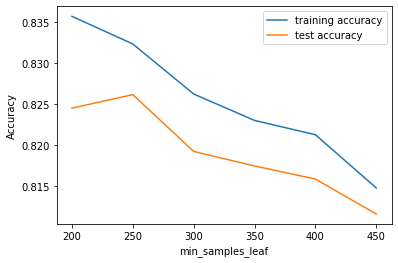##### Tuning max features
In :
``````# GridSearchCV to find optimal min_samples_split
from sklearn.model_selection import KFold
from sklearn.model_selection import GridSearchCV

# specify number of folds for k-fold CV
n_folds = 5

# parameters to build the model on
parameters = {'max_features': range(5, 30, 5)}

# instantiate the model
rf = RandomForestClassifier()

# fit tree on training data
rf = GridSearchCV(rf, parameters,
cv=n_folds,
scoring="accuracy",return_train_score=True)
rf.fit(Xtrain_reduced, y_train)
``````
Out:
``````GridSearchCV(cv=5, error_score='raise-deprecating',
estimator=RandomForestClassifier(bootstrap=True, class_weight=None,
criterion='gini', max_depth=None,
max_features='auto',
max_leaf_nodes=None,
min_impurity_decrease=0.0,
min_impurity_split=None,
min_samples_leaf=1,
min_samples_split=2,
min_weight_fraction_leaf=0.0,
n_estimators='warn', n_jobs=None,
oob_score=False,
random_state=None, verbose=0,
warm_start=False),
iid='warn', n_jobs=None,
param_grid={'max_features': range(5, 30, 5)},
pre_dispatch='2*n_jobs', refit=True, return_train_score=True,
scoring='accuracy', verbose=0)``````
In :
``````# scores of GridSearch CV
scores = rf.cv_results_
pd.DataFrame(scores).head()
``````
Out:
In :
``````# plotting accuracies with min_samples_split
plt.figure()
plt.plot(scores["param_max_features"],
scores["mean_train_score"],
label="training accuracy")
plt.plot(scores["param_max_features"],
scores["mean_test_score"],
label="test accuracy")
plt.xlabel("max_features")
plt.ylabel("Accuracy")
plt.legend()
plt.show()
``````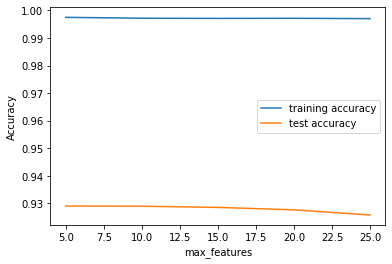#### Grid Search to Find Optimal Hyperparameters

We can now find the optimal hyperparameters using GridSearchCV.

In :
``````from sklearn.model_selection import KFold
from sklearn.model_selection import GridSearchCV

# Create the parameter grid based on the results of random search
param_grid = {
'max_depth': [12,14,16],
'min_samples_leaf': range(100, 200, 350),
'min_samples_split': range(100, 150, 200),
'n_estimators': [30,60, 90],
'max_features': [10, 15]
}
# Create a based model
rf = RandomForestClassifier()
# Instantiate the grid search model
grid_search = GridSearchCV(estimator = rf, param_grid = param_grid,
cv = 3, n_jobs = -1,verbose = 1)``````
In :
``````# Fit the grid search to the data
grid_search.fit(Xtrain_reduced, y_train)
``````
```Fitting 3 folds for each of 18 candidates, totalling 54 fits ```
```[Parallel(n_jobs=-1)]: Using backend LokyBackend with 4 concurrent workers. [Parallel(n_jobs=-1)]: Done 42 tasks | elapsed: 5.3min [Parallel(n_jobs=-1)]: Done 54 out of 54 | elapsed: 7.3min finished ```
Out:
``````GridSearchCV(cv=3, error_score='raise-deprecating',
estimator=RandomForestClassifier(bootstrap=True, class_weight=None,
criterion='gini', max_depth=None,
max_features='auto',
max_leaf_nodes=None,
min_impurity_decrease=0.0,
min_impurity_split=None,
min_samples_leaf=1,
min_samples_split=2,
min_weight_fraction_leaf=0.0,
n_estimators='warn', n_jobs=None,
oob_score=False,
random_state=None, verbose=0,
warm_start=False),
iid='warn', n_jobs=-1,
param_grid={'max_depth': [12, 14, 16], 'max_features': [10, 15],
'min_samples_leaf': range(100, 200, 350),
'min_samples_split': range(100, 150, 200),
'n_estimators': [30, 60, 90]},
pre_dispatch='2*n_jobs', refit=True, return_train_score=False,
scoring=None, verbose=1)``````
In :
``````# printing the optimal accuracy score and hyperparameters
print('We can get accuracy of',grid_search.best_score_,'using',grid_search.best_params_)``````
```We can get accuracy of 0.8483205836161913 using {'max_depth': 14, 'max_features': 10, 'min_samples_leaf': 100, 'min_samples_split': 100, 'n_estimators': 60} ```

Fitting the final model with the best parameters obtained from grid search.

In :
``````# model with the best hyperparameters
from sklearn.ensemble import RandomForestClassifier
rfc_selected_model = RandomForestClassifier(bootstrap=True,
max_depth=16,
min_samples_leaf=100,
min_samples_split=100,
max_features=10,
n_estimators=90)``````
In :
``````# fit
rfc_selected_model.fit(Xtrain_reduced,y_train)
``````
Out:
``````RandomForestClassifier(bootstrap=True, class_weight=None, criterion='gini',
max_depth=16, max_features=10, max_leaf_nodes=None,
min_impurity_decrease=0.0, min_impurity_split=None,
min_samples_leaf=100, min_samples_split=100,
min_weight_fraction_leaf=0.0, n_estimators=90,
n_jobs=None, oob_score=False, random_state=None,
verbose=0, warm_start=False)``````
In :
``````# predictions for the selected model
predictions_final_selected = rfc_selected_model.predict(Xtest_reduced)
``````
In :
``````# evaluation metrics
from sklearn.metrics import classification_report,confusion_matrix,accuracy_score``````
In :
``print(classification_report(y_test,predictions_final_selected))``
``` precision recall f1-score support 0 0.97 0.86 0.91 4910 1 0.37 0.75 0.49 517 accuracy 0.85 5427 macro avg 0.67 0.81 0.70 5427 weighted avg 0.91 0.85 0.87 5427 ```
In :
``print(confusion_matrix(y_test,predictions_final_selected))``
```[[4243 667] [ 128 389]] ```
In :
``print(accuracy_score(y_test,predictions_final_selected))``
```0.853510226644555 ```

We see that on tuning the hyperparameters we get a accuracy of `85.42 %` , precision of `67%` , recall of `81%`.

#### SVM Model with PCA Components

In :
``from sklearn.svm import SVC``
In :
``````# Model building

# instantiate an object of class SVC()
# note that we are using cost C=1
svm_model = SVC(C = 1)

# fit
svm_model.fit(Xtrain_reduced, y_train)

# predict
y_pred = svm_model.predict(Xtest_reduced)
``````
In :
``````# Evaluate the model using confusion matrix
from sklearn import metrics
metrics.confusion_matrix(y_true=y_test, y_pred=y_pred)
``````
Out:
``````array([[4513,  397],
[ 186,  331]], dtype=int64)``````
In :
``````# print other metrics

# accuracy
print("accuracy", metrics.accuracy_score(y_test, y_pred))

# precision
print("precision", metrics.precision_score(y_test, y_pred))

# recall/sensitivity
print("recall", metrics.recall_score(y_test, y_pred))

``````
```accuracy 0.892574166206007 precision 0.45467032967032966 recall 0.6402321083172147 ```

The SVM we have built so far gives decently moderate results - an accuracy of 89%, sensitivity/recall (TNR) of 64%.

## Interpretation of Results

89% of all churn are classified correctly 64% of churn are identified correctly (sensitivity/recall) Specificity, or % of non-churn classified correctly, is 45.4%

#### K-Fold Cross Validation

Let's first run a simple k-fold cross validation to get a sense of the average metrics as computed over multiple folds. the easiest way to do cross-validation is to use the `cross_val_score()` function.

In :
``````# creating a KFold object with 5 splits
folds = KFold(n_splits = 5, shuffle = True, random_state = 4)

# instantiating a model with cost=1
svm_model_tuned = SVC(C = 1)``````
In :
``````from sklearn.model_selection import cross_val_score
# computing the cross-validation scores
# note that the argument cv takes the 'folds' object, and
# we have specified 'accuracy' as the metric

cv_results = cross_val_score(svm_model_tuned, Xtrain_reduced, y_train, cv = folds, scoring = 'accuracy') ``````
In :
``````# print 5 accuracies obtained from the 5 folds
print(cv_results)
print("mean accuracy = {}".format(cv_results.mean()))``````
```[0.94224924 0.94136272 0.94034954 0.94528182 0.93742875] mean accuracy = 0.9413344151615075 ```

Thus , we see that the mean accuracy of the svm model is `0.94`
Precision with default_parameters is `0.45`
Recall with default parameters is `0.64`

##### XG Boost Model with PCA Components
In :
``````! pip install xgboost
from xgboost import XGBClassifier``````
```Collecting xgboost Downloading https://files.pythonhosted.org/packages/5e/49/b95c037b717b4ceadc76b6e164603471225c27052d1611d5a2e832757945/xgboost-0.90-py2.py3-none-win_amd64.whl (18.3MB) Requirement already satisfied: scipy in c:\users\arijit das\anaconda3\lib\site-packages (from xgboost) (1.3.1) Requirement already satisfied: numpy in c:\users\arijit das\anaconda3\lib\site-packages (from xgboost) (1.16.5) Installing collected packages: xgboost Successfully installed xgboost-0.90 ```
In :
``xgb_model = XGBClassifier()``
In :
``xgb_model.fit(Xtrain_reduced,y_train)``
Out:
``````XGBClassifier(base_score=0.5, booster='gbtree', colsample_bylevel=1,
colsample_bynode=1, colsample_bytree=1, gamma=0,
learning_rate=0.1, max_delta_step=0, max_depth=3,
min_child_weight=1, missing=None, n_estimators=100, n_jobs=1,
nthread=None, objective='binary:logistic', random_state=0,
reg_alpha=0, reg_lambda=1, scale_pos_weight=1, seed=None,
silent=None, subsample=1, verbosity=1)``````
In :
``y_pred = xgb_model.predict(Xtest_reduced)``
In :
``accuracy_score(y_pred,y_test)``
Out:
``0.8395061728395061``
In :
``precision_score(y_pred,y_test)``
Out:
``0.781431334622824``
In :
``recall_score(y_pred,y_test)``
Out:
``0.3476764199655766``

From , XG Boost Classifier, we get the following metrics for the model with PCA Components:

1. Accuracy Score - `83.9%`
2. Precision Score - `78.1%`
3. Recall Score - `34.76%`

Thus from the models we see above with PCA , we see that random forest has performed best in terms of recall and precision score.

Recall is important here, as it gives a measure of the predicted customers to churn against customers who would actually churn. Since the company focuses on retaining the high-value customers, so high recall for the model is important. On the other hand, precision is a measure of the actual customers who would churn out, among those predicted to churn out. So, even if the model predicts few customers to churn out eventhough they will not churn actually, that is less harmful than the model not able to identify a customer who would actually churn out.

In :
``final_pred = pd.Series(predictions_final_selected)``
In :
``final_pred.value_counts()``
Out:
``````0    4371
1    1056
dtype: int64``````

Thus `19.4` % of the high-value customers are predicted to churn in the test data.

From the ridge regression model we see that the top 5 columns having being assigned the highest coefficients are :
1.`total_rech_data_8` --> Total recharge of data for the month of august

2. `loc_ic_t2f_mou_8` --> minutes of local incoming calls within same circle within fixed lines inside same operator
3. `loc_ic_t2m_mou_8` --> minutes of local incoming calls within same circle within fixed lines to other operator

1. `loc_ic_t2t_mou_8` -->minutes of local incoming calls within same circle inside same operator through mobile

2. `std_og_mou_8` --> Minutes of usage of standard outgoing voice calls in month of august

In :
``df_feature_selected = df_ridge_feature_select.loc[df_ridge_feature_select['coefficient'].isin(top5features)]``
In :
``````x= df_feature_selected['coefficient'].values
y= df_feature_selected.index
``````
In :
``````sns.barplot(x=x,y=y)
plt.title('importance of features for predicting churn')
plt.show()

``````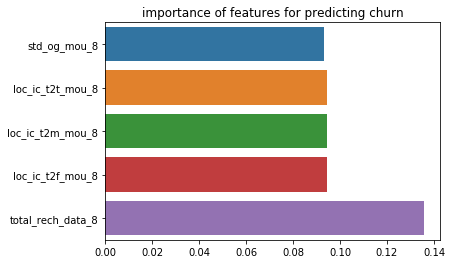#### Business Recommendation:

Company should mainly focus on data recharge done by customers in the action phase , they should give higher data friendly packs to customers who are about to churn. Also, they should improve on the inter network calls within their circle both on telephone lines and mobile for customers who might churn.

In [ ]:
`` ``